## 1 Introduction

The task of assessing similarity between two nodes based on graph topology is a long-standing problem in hyperlink analysis. This type of similarity, also known as link-based similarity, is one of the fundamental primitives in a broad range of applications, e.g., recommendation systems , web page ranking , spam detection , citation analysis , and graph clustering . Indeed, link-based similarity relies on graph structures to assess relevance between two nodes, in contrast to text-based similarity that hinges on the text content of the Web. However, it is a complex challenge to find an effective and scalable link-based similarity model since a desirable similarity measure should not only better simulate human judgement behavior based on simple and elegant formulations , but also scale well on large graphs.

Recently, SimRank  has received growing interest as a widely-accepted measure of pairwise similarity. The triumph of SimRank is largely due to its succinct yet elegant idea that “two nodes are assessed as similar if they are pointed to by similar nodes”, together with the base case that “each node is most similar to itself”. SimRank was first proposed by Jeh and Widom , and has gained tremendous popularity in many vibrant communities, e.g., collaborative filtering , social network analysis , and k-nearest neighbor search . Since then, there has also been some studies [10, 11, 19, 33] focusing on Li et al. ’s SimRank model , a variant of Jeh and Widom’s model. The recent studies [16, 34] show the difference between these two SimRank models: In Jeh and Widom’s model , the SimRank similarity of each node with itself is always 1, whereas in Li et al. ’s model  there is no such a restriction. However, due to the self-referentiality, both SimRank models suffer from high computational overhead.

While significant efforts have been devoted to optimizing computation of both SimRank models [9,10,11, 16, 19, 24, 26, 27, 32, 33], semantic issues of SimRank have attracted little attention. We observe that both SimRank models have an undesirable property (we call it “zero-similarity”): SimRank score s(ij) only accommodates the paths of equal length from a common “source” node to both i and j, but other paths for node-pair (ij) are fully ignored by SimRank, as shown in Example 1.

### Example 1

Consider a citation network $${{{\mathcal {G}}}}$$ in Fig. 1, where each node is a paper, and an edge is a citation. Given damping factor $$C=0.6$$, query node f, and the number of iterations $$K=20$$, we assess all SimRank similarities $$\{s(\star ,f)\}$$ w.r.t. query f in $${{\mathcal {G}}}$$, using both Jeh and Widom’s model  and Li et al. ’s model , whose results are shown in columns JSR and LSR, respectively. We notice that, regardless of which SimRank model is used, many node-pairs in $${\mathcal {G}}$$ have zero similarities when they have no incoming paths with equal length from a common “source” node. For instance, $$s(e,f)=0$$ since the in-link “source” a is not in the center of the path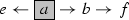. This means that when we recursively compute the pairwise in-neighborhood similarities of two nodes, there is no likelihood for this recursion to reach the base case (i.e., a common in-link “source”) that a node is maximally similar to itself. Similarly, $$s(a,f)=0$$ since a has no in-neighbors, not to mention the fact that there is no such a common in-link “source” with equal distance to both a and f. In contrast, $$s(c,f)>0$$ since there is a common in-link “source” b in the center of the path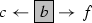. $$\square$$

The “zero-SimRank” phenomenon in Example 1 is rather counter-intuitive, e.g., $$s(e,f)=0$$. We note from Fig. 1 that e and f do have a common in-link “source” a, just except for the equal-length distance from a to both e and f. Hence, e and f should have some relevance. Another example is a path graph of length 2n: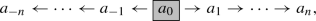where each $$a_{i} \ (i=0,\pm 1,\ldots , \pm n)$$ denotes a node. We notice that SimRank score $$s(a_i,a_j)=0$$, for all $$|i| \ne |j|$$, which is quite against intuition since $$a_{0}$$ is the common root of all nodes $$a_i \ (i=\pm 1,\ldots , \pm n)$$.

It is important to notice that the “zero-similarity” issue refers to not only the problem that SimRank may produce “complete zero scores” (i.e., “completely dissimilar” issue), but also the problem that SimRank will neglect the contributions of a large class of in-link paths whose “source” node is not in the center (even though their similarity scores are not zero) due to the “zero contributions” of such paths to SimRank scores (i.e., “partially missing” issue). Indeed, as demonstrated by our experiments in Fig. 6b, both issues of “zero-similarity” commonly exist in real graphs, e.g., on CitH, $$\sim \,97.9\%$$ node-pairs have “zero-SimRank” issues, among which $$\sim \,19.2\%$$ are evaluated to be “completely dissimilar”, and $$\sim \,78.7\%$$ (though SimRank $$\ne$$0) to be “partially missing” the contributions of many in-link paths. These have adversely affected assessment quality, which highlights our need to enhance the existing SimRank model.

A pioneering piece of work by Zhao et al.  proposes rudiments of a novel approach to refining the SimRank model. Observing that SimRank may incur some unwanted “zero similarities”, they suggested P-Rank, an extension of SimRank, by taking both in- and out-links into consideration for similarity assessment, as opposed to SimRank that merely considers in-links. Although P-Rank, to some degree, might reduce “zero-similarity” occurrences in practice, we argue that such a “zero-similarity” issue arises, not because of a biased overlook of SimRank against out-links, but because of the blemish in SimRank philosophy that may miss the contribution of a certain kind of paths (whose in-link “source” is not in the center). In other words, P-Rank cannot, in essence, resolve the “zero-similarity” issue of SimRank. For instance, nodes a and f are similar in the context of P-Rank, as shown in column PR of Fig. 1, since there is an out-link “source” d in the center of the outgoing path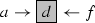. However, the P-Rank similarity of (ef) is still zero, since (1) i is not in the center of the outgoing path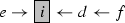, and (2) there are no other outgoing paths between pair (ef).

Our main goal in this work is to propose an effective and scalable model that remedies the “zero-similarity” issue of SimRank, while capturing merits of the original SimRank philosophy. Keeping with an elegant form and supporting scalability on large graphs, our model is intended to be an enhancement of SimRank for semantic richness, and takes into account contributions of many incoming paths (whose common “source” is not strictly in the center) that are neglected by SimRank. A major challenge with establishing this model is that it is notoriously difficult to effectively assess s(ab) by finding out all the possible incoming paths between a and b, regardless of whether there exists a common “source” with equal distance to both a and b. Fortunately, we observe that our model can be reduced to a simple elegant closed form, without suffering from high computational time and memory space. Our proposed model can handle all-pairs similarities query, and we are more interested in the single-source query, i.e.,

Given :

a graph $${\mathcal {G}}$$, and a query node q in $${\mathcal {G}}$$

Retrieve :

all the similarities $$\{s(\star ,q)\}$$ w.r.t. query q according to our proposed similarity measure.

This type of query is practically useful when answering the questions such as “who have close interactions with Diego (query) in a social network?”, and “which papers are relevant to this one (query) in a co-citation graph?”.

### 1.1 Main contributions

• We first provide a sufficient and necessary condition of the “zero-similarity” problem for the existing similarity models, e.g., Jeh and Widom’s SimRank , Li et al. ’s SimRank , Random Walk with Restart (RWR) , and ASCOS++  (Sect. 3).

• We propose SimRank*, a semantic enhanced version of SimRank, and explain its semantic richness. Our model provides a way of traversing more incoming paths that are largely ignored by SimRank, and thus enables counter-intuitive “zero-SimRank” nodes to be similar while inheriting the beauty of the SimRank philosophy (Sect. 4).

• We convert the series form of SimRank* to a closed form, which looks more succinct yet with richer semantics than SimRank, without suffering from increased computational cost. This leads to an iterative model for computing all-pairs SimRank* in O(Knm) time and $$O(n^2)$$ memory on a graph of n nodes and m edges for K iterations (Sect. 5).

• To speed up SimRank* computation further, as the existing technique  of partial sums memoization for SimRank optimization no longer applies, we leverage a novel clustering approach via edge concentration. Due to its NP-hardness, an efficient algorithm is devised to speed up all-pairs SimRank* computation to $$O(K n {\tilde{m}})$$ time, where $${\tilde{m}}$$ is the number of edges in our compressed graph, which is generally much smaller than m (Sect. 6).

• To scale SimRank* over billion-edge graphs, we also propose two memory-efficient single-source algorithms for SimRank*, i.e., ss-gSR* for geometric SimRank*, and ss-eSR* for exponential SimRank*, that require $$O(K^2 {\tilde{m}})$$ time and $$O(K {\tilde{m}})$$ time, respectively, to compute similarities between all n nodes and a given query on an as-needed basis. This significantly reduces the $$O(n^2)$$ memory of all-pairs search to either $$O(Kn + {\tilde{m}})$$ for geometric SimRank*, or $$O(n + {\tilde{m}})$$ for exponential SimRank*, without any compromise of accuracy, where $${\tilde{m}} \ll n^2$$ (Sect. 7).

• We also compare SimRank* with another alternative remedy for SimRank that adds self-loops on each node, and demonstrate that SimRank* is more effective (Sect. 8).

• We evaluate the performance of SimRank* on real and synthetic datasets. Empirical results show that (i) SimRank* achieves richer semantics than existing measures (e.g., SimRank, P-Rank, and RWR); (ii) Our optimization techniques for SimRank* are consistently faster than the baselines by several times; (iii) SimRank* is scalable on large graphs with billions of edges, without any compromise of accuracy; (iv) The impacts of the query size and the number of iterations on the time and memory performance of SimRank* over large-scale graphs (Sect. 9).

• In Sects. 3.2 and 3.5, we provide a sufficient and necessary condition of the “zero-similarity” problem for Jeh and Widom’s SimRank model  and ASCOS++ (a RWR-like model that appeared recently) . In contrast, the prior work  only focused on Li et al. ’s SimRank model . However, recent studies [16, 34] have pointed out that these two SimRank models are different. Thus, it is imperative to investigate if the similar “zero-similarity” problem exists in Jeh and Widom’s SimRank model. Moreover, in Sect. 3.3, we add Corollary 2 to show that the positions of node-pairs with “zero-similarity” issues in both SimRank models are exactly the same.

• In Sect. 7, we propose two memory-efficient SimRank* single-source algorithms, ss-gSR* and ss-eSR*, that support on-demand computation of similarities between all n nodes and a given query in $$O(K^2{\tilde{m}})$$ time and $$O(K{\tilde{m}})$$ time, respectively. These algorithms also significantly reduce the space of all-pairs SimRank* from $$O(n^2)$$ to $$O(Kn+{\tilde{m}})$$ for geometric SimRank* search, and to $$O(n+{\tilde{m}})$$ for exponential SimRank* search, respectively, without any sacrifice of accuracy. We also provide the complexity bounds and correctness proofs of our memory-efficient algorithms. This has made the previous version of the SimRank* model in  highly scalable to large graphs with billions of edges.

• In Sect. 8, we compare SimRank* with another alternative remedy of SimRank that adds self-loops on each node. Our analysis demonstrate that SimRank* is more effective than the straightforward treatment of adding self-loops, since SimRank* does not repeatedly count any incoming paths of different length when assessing pairwise similarity.

• In Sects. 9.2.2 and 9.2.39.2.5, we conduct additional experiments on a variety of large-scale datasets, including (i) qualitative case studies of the rich semantics of SimRank* for single-source queries on real labeled datasets (DBLP and CitH); (ii) high scalability and low computational cost in terms of time and space for our memory-efficient SimRank* algorithms over billion-edge graphs; (iii) exactness of ss-gSR* and ss-eSR* as compared with the previous algorithms proposed in ; and (iv) impacts of the size of queries |Q| and the number of iterations K on the time and memory of ss-gSR* and ss-eSR* on large-scale datasets.

• In Sect. 10, we update related work by incorporating the new research that has appeared recently.

## 2 Preliminaries

In this section, we revisit the background of SimRank. Previous studies on SimRank can be distinguished into two categories, based on the SimRank model they used: (1) Jeh and Widom’s model (e.g., [9, 12, 16, 24, 25, 27]) and (2) Li et al. ’s model (e.g., [10, 11, 19, 33, 39]). Recent works [16, 34] have pointed out that two SimRank models are different. Let us look at the component form and matrix form of each SimRank model, respectively. Table 1 lists the notations frequently used in the article.

### 2.1 Jeh and Widom’s SimRank model

Let $$\mathcal {G}=(\mathcal {V},\mathcal {E})$$ be a given graph with a set of nodes, $$\mathcal {V}$$, and a set of edges, $$\mathcal {E}$$. We denote by $$\mathcal {I} (a)$$ a set of all the in-neighbors of a, and $$| \mathcal {I} (a) |$$ the cardinality of $$\mathcal {I} (a)$$.

Component formJeh and Widom’s SimRank score between nodes a and b, denoted as s(ab), is defined as

1. (i)

$$s(a,b)=0$$, if $${\mathcal {I}}(a)=\varnothing \text { or } {\mathcal {I}}(b)=\varnothing$$;

2. (ii)

otherwise,(1)

where $$C \in (0,1)$$ is a damping factor.

Iterative form To iteratively solve s(ab), Jeh and Widom  carried out the following iterations:

1. (i)

Start with $$s_0 (a,a)=1$$ and $$s_0(a,b)=0$$ if $$a \ne b$$.

2. (ii)

For $$k=0,1,2,\ldots$$, iterate as indicated below:

1. (a)

$$s_{k+1}( a,b )=0$$, if $${\mathcal {I}}\left( a \right) =\varnothing \text { or } {\mathcal {I}}\left( b \right) =\varnothing$$;

2. (b)

otherwise,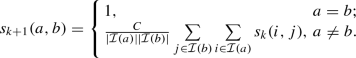(2)

The resulting $${\{s_k(a,b)\}}_{k=0}^{\infty }$$ converges to s(ab).

Matrix form Recently, Kusumoto et al.  have provided the matrix form for Jeh and Widom’s SimRank model, which is equivalent to Eq. (1):

\begin{aligned} {{{\mathbf {S}}}}= \max \{ C \cdot (\mathbf {Q} \cdot {{{\mathbf {S}}}}\cdot {{{\mathbf {Q}}}^{T}} ), {{{\mathbf {I}}}_{n}} \}, \end{aligned}
(3)

where $${\mathbf {S}}$$ is Jeh and Widom’s similarity matrix whose entry $$[{\mathbf {S}}]_{i,j}$$ is SimRank score s(ij); $${\mathbf {Q}}$$ is the backward transition matrix with its entry $$[{\mathbf {Q}}]_{i,j}$$ defined as

\begin{aligned} {[}{\mathbf {Q}}]_{i,j} = \left\{ \begin{array}{ll} 1/|\mathcal {I}(i)|, &{} \text { if }\exists \text { edge }(j \rightarrow i) \in {\mathcal {E}}; \\ 0, &{} \text {otherwise.} \end{array} \right. \end{aligned}

$${\mathbf {Q}}^\mathrm{T}$$ is the matrix transpose of $${\mathbf {Q}}$$; $$\max \{\cdot \}$$ is the element-wise maximum operator; $${\mathbf {I}}_n$$ is an $$n \times n$$ identity matrix.

### 2.2 Li et al. ’s SimRank model

To differentiate Jeh and Widom’s SimRank matrix $${\mathbf {S}}$$, we use $${{\mathbf {S_L}}}$$ to denote Li et al. ’s SimRank matrix. The matrix form of Li et al. ’s SimRank model  is

\begin{aligned} {{\mathbf {S_L}}}= C\cdot ({\mathbf {Q}} \cdot {{\mathbf {S_L}}}\cdot {{{\mathbf {Q}}}^{T}} ) + (1-C) \cdot {{{\mathbf {I}}}_{n}}, \end{aligned}
(4)

It is worth noticing that the term $$(1-C) \cdot {{{\mathbf {I}}}_{n}}$$ in Eq. (4) cannot guarantee that all diagonal values of $$\mathbf {S_L}$$ are 1s, which is different to Jeh and Widom’s model in Eq. (3).

Accordingly, Eq. (4) can be readily rewritten into the following component form:

(i) $$s_L(a,b)=0$$, if $${\mathcal {I}}(a)=\varnothing \text { or } {\mathcal {I}}(b)=\varnothing$$;

(ii) otherwise,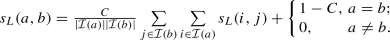## 3 “Zero-similarity” problem

In this section, we will provide a sufficient and necessary condition of the “zero-similarity” problem for Jeh and Widom’s SimRank , Li et al. ’s SimRank , RWR , and ASCOS .

Before illustrating the existence of “zero-Similarity” problems, let us first introduce the following notions.

### Definition 1

(An In-Link Path) An in-link path$$\rho$$ of node-pair (ab) in $${{\mathcal {G}}}$$ is a walk of length $$(l_1 + l_2)$$, denoted as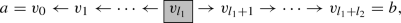starting from node a, taking $$l_1$$ steps against the directions of the edges $$v_{i-1} \leftarrow v_i$$ for every $$i \in [1,l_1]$$, and $$l_2$$ steps along the directions of $$v_{i-1} \rightarrow v_{i}$$ for every $$i\in [l_1+1,l_1+l_2]$$, and finally arriving at node b. Here, node $$v_{l_1}$$ is called the in-link “source” of $$\rho$$; and the length of in-link path $$\rho$$, denoted by $$\textsf {len}(\rho )$$, is $$(l_1 + l_2)$$, i.e., the number of edges in $$\rho$$. We allow a path from the “source” node to one end with repeated nodes to suit the existence of cycles in a graph.

### Definition 2

An in-link path $$\rho$$ is called symmetric if $$l_1=l_2$$. $$\rho$$ is called unidirectional if $$l_1=0$$ or $$l_2=0$$.

### Example 2

Consider the graph $${\mathcal {G}}$$ in Fig. 1, the path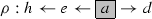is an in-link path of node-pair (hd), where a is the in-link “source”. $$\textsf {len}(\rho )=2+1=3$$. $$\rho$$ is an asymmetric in-link path as $$l_1=2\ne 1=l_2$$. $$\square$$

Clearly, an in-link path $$\rho$$ is symmetric if and only if there exists an in-link “source” in the center of $$\rho$$. Thus, any in-link path of odd length (i.e., $$l_1+l_2$$ is odd) is asymmetric since there do not exist two integers $$l_1$$ and $$l_2$$ s.t. $$l_1=l_2$$ and $$l_1 +l_2$$ is odd.

To count the number of the in-link paths in a graph $${\mathcal {G}}$$, we extend the power property of an adjacency matrix.

Traditionally, let $${\mathbf {A}}$$ be the adjacency matrix of $${\mathcal {G}}$$. There is an interesting property of $${\mathbf {A}}^l$$ : The entry $${[{{\mathbf {A}}}^{l}]}_{i,j}$$ counts the number of paths of length l from node i to j. This property can be generalized as follows:

### Lemma 1

Let $$\rho$$ be a generic path of length l that consists of a sequence of nodes $$i=v_0,v_1,\ldots ,v_l=j$$, where each edge can be directed either a) from $$v_{k-1}$$ to $$v_k$$, or b) from $$v_k$$ to $$v_{k-1}$$. Let $$\bar{{\mathbf {A}}}={\prod }_{k=1}^{l} {\mathbf {A}}_k$$, where

\begin{aligned} {\mathbf {A}}_k = \left\{ \begin{array}{ll} {\mathbf {A}}, &{} \text { if }\exists \text { an edge }(v_{k-1} \rightarrow v_k)\text { in }\rho \\ {\mathbf {A}}^\mathrm{T}, &{} \text { if }\exists \text { an edge }(v_{k-1} \leftarrow v_k)\text { in }\rho \end{array} \right. , \ \text { for } k\in [1,l] \end{aligned}

Then, $${[\bar{{\mathbf {A}}}]}_{i,j}$$ counts the number of generic paths $$\rho$$ in $$\mathcal {G}$$.

The proof of Lemma 1 is completed by induction on l, which is similar to the proof of the power property of the adjacency matrix [5, Page 51].

Intuitively, Lemma 1 counts the number of generic paths whose edges are not always in the same direction. For instance, consider a path $$\rho : i \rightarrow \circ \leftarrow \circ \rightarrow \circ \rightarrow \circ \leftarrow j$$, where $$\circ$$ denotes an arbitrary node in a graph. We can construct $$\bar{{\mathbf {A}}}={\mathbf {A}} {\mathbf {A}}^\mathrm{T} {\mathbf {A}} {\mathbf {A}} {\mathbf {A}}^\mathrm{T}$$, where $${\mathbf {A}}$$ (resp. $${\mathbf {A}}^\mathrm{T}$$) is at the positions 1, 3, 4 (resp. 2, 5), corresponding to the positions of $$\rightarrow$$ (resp. $$\leftarrow$$) in $$\rho$$. Then, $${[\bar{{\mathbf {A}}}]}_{i,j}$$ tallies the number of paths $$\rho$$ in the graph. If no such paths, $${[\bar{{\mathbf {A}}}]}_{i,j}=0$$. As another example, $${[{({\mathbf {A}}^\mathrm{T})}^{l_1} \cdot {\mathbf {A}}^{l_2}]}_{i,j}$$ tallies the number of in-link paths of length $$(l_1+l_2)$$ for node-pair (ij). As a special case when all $${\mathbf {A}}_k \ (\forall k\in [1,l])$$ are set to $${\mathbf {A}}$$, Lemma 1 reduces to the conventional power property of an adjacency matrix.

An immediate consequence of Lemma 1 is as follows:

### Corollary 1

$$\sum _{k=1}^{\infty }{[{({\mathbf {A}}^\mathrm{T})}^{k} \cdot {\mathbf {A}}^{k}]}_{i,j}$$ counts the number of all symmetric in-link paths of node-pair (ij) in $${\mathcal {G}}$$.

Corollary 1 implies that if there are no nodes with equal distance to both i and j (i.e., if no symmetric in-link paths for node-pair (ij)), then

\begin{aligned} {[{({\mathbf {A}}^\mathrm{T})}^{k} \cdot {\mathbf {A}}^{k}]}_{i,j} =0, \qquad (\forall k=1,2,\ldots ) \end{aligned}

### 3.2 “Zero-similarity” issue in Jeh and Widom’s model

Based on the notions of symmetric in-link paths, we next show why the “zero-similarity” issue exists in Jeh and Widom’s model. Specifically, we show the following theorem:

### Theorem 1

For any two distinct nodes a and b in $${\mathcal {G}}$$, Jeh and Widom’s SimRank score s(ab) will ignore all the contributions of asymmetric in-link paths for (ab). As an extreme case, $$s(a,b)=0$$ if and only if there are no symmetric in-link paths in $${\mathcal {G}}$$ for node-pair (ab).

### Proof

Let $$diag({\mathbf {X}})$$ be a matrix operator that returns a diagonal matrix whose diagonal entries are the same as the matrix $${\mathbf {X}}$$. Then, Jeh and Widom’s SimRank Eq. (3) can be rewritten as:

\begin{aligned} {\mathbf {S}}=C\cdot ({\mathbf {Q}}\cdot {\mathbf {S}}\cdot {{{\mathbf {Q}}}^{T}})+{\mathbf {D}} \end{aligned}
(5)

where $${\mathbf {D}}={{{\mathbf {I}}}_{n}}-C\cdot diag({\mathbf {Q}}\cdot {\mathbf {S}}\cdot {{{\mathbf {Q}}}^{T}})$$ is a diagonal matrix.

It is important to notice that each diagonal element $$[{\mathbf {D}}]_{i,i} \in [1-C, 1]$$. This is because

\begin{aligned} \textstyle {{[{\mathbf {D}}]}_{i,i}}=1-C\cdot \sum _{x=1}^{n}{\sum _{y=1}^{n}{{{[{\mathbf {Q}}]}_{i,x}}\cdot {{[{\mathbf {S}}]}_{x,y}}}\cdot {{[{\mathbf {Q}}]}_{i,y}}} \end{aligned}

Since $$0\le {{[{\mathbf {S}}]}_{x,y}}\le 1$$ and $$0 \le \sum _{x=1}^{n} {{[{\mathbf {Q}}]}_{i,x}} \le 1$$, we have

\begin{aligned} \textstyle {{[{\mathbf {D}}]}_{i,i}}\ge 1-C\cdot \sum _{x=1}^{n}{{{[{\mathbf {Q}}]}_{i,x}}\cdot \sum _{y=1}^{n}{{{[{\mathbf {Q}}]}_{i,y}}}} \ge 1-C \end{aligned}

According to Kusumoto et al. , Eq. (5) takes the following power series form:

\begin{aligned} \textstyle {\mathbf {S}}= \sum _{l=0}^{\infty } C^l \cdot {\mathbf {Q}}^l \cdot {\mathbf {D}} \cdot {({\mathbf {Q}}^\mathrm{T})}^l, \end{aligned}

whose component form is

\begin{aligned} \textstyle [{\mathbf {S}}]_{i,j}= \sum _{l=0}^{\infty } C^l \cdot [{\mathbf {Q}}^l \cdot {\mathbf {D}} \cdot {({\mathbf {Q}}^\mathrm{T})}^l]_{i,j} \end{aligned}
(6)

We next show that $$[{\mathbf {S}}]_{i,j} \ne 0$$ whenever there exists a symmetric in-link path for node-pair (ij).

(Sufficiency) We first prove that

$$\exists$$ a symmetric in-link path for $$(i,j) \ \Rightarrow \ [{\mathbf {S}}]_{i,j} \ne 0$$”.

If there exists a symmetric in-link path for (ij), then there exists a node $$x_0$$ in the center of this in-link path, such that the symmetric in-link path can be divided into two unidirectional paths of equal length $$l_0$$:

\begin{aligned} \underbrace{i \leftarrow \circ \leftarrow \cdots \leftarrow \circ \leftarrow x_0}_{\text {length } l_0} \ \text { and } \ \underbrace{x_0 \rightarrow \circ \rightarrow \cdots \rightarrow \circ \rightarrow j}_{\text {length } l_0} \end{aligned}

Thus, by Lemma 1, it follows that

\begin{aligned}&[({\mathbf {A}}^\mathrm{T})^{l_0}]_{i,x_0} \ne 0&\text { and } [{\mathbf {A}}^{l_0}]_{x_0,j} \ne 0 \\&\quad \Leftrightarrow [{\mathbf {Q}}^{l_0}]_{i,x_0}> 0&\text { and } [({\mathbf {Q}}^\mathrm{T})^{l_0}]_{x_0,j} > 0 \end{aligned}

Since each term $$[{\mathbf {Q}}^l \cdot {\mathbf {D}} \cdot {({\mathbf {Q}}^\mathrm{T})}^l]_{i,j} \ \ (\forall l)$$ in Eq. (6) is nonnegative, we have

\begin{aligned} \textstyle [{\mathbf {S}}]_{i,j}&\ge C^{l_0} \cdot [{\mathbf {Q}}^{l_0} \cdot {\mathbf {D}} \cdot {({\mathbf {Q}}^\mathrm{T})}^{l_0}]_{i,j} \\&\textstyle = C^{l_0} \cdot \sum _{x,y} [{\mathbf {Q}}^{l_0}]_{i,x} \cdot [{\mathbf {D}}]_{x,y} \cdot [{({\mathbf {Q}}^\mathrm{T})}^{l_0}]_{y,j} \\&\ge C^{l_0} \cdot [{\mathbf {Q}}^{l_0}]_{i,x_0} \cdot [{\mathbf {D}}]_{x_0,x_0} \cdot [{({\mathbf {Q}}^\mathrm{T})}^{l_0}]_{x_0,j} \end{aligned}

Since $$C^{l_0}>0$$, $$[{\mathbf {Q}}^{l_0}]_{i,x_0} > 0$$, $$[({\mathbf {Q}}^\mathrm{T})^{l_0}]_{x_0,j} > 0$$, and $$[{\mathbf {D}}]_{x_0,x_0} \ge 1-C>0$$, it follows that $$[{\mathbf {S}}]_{i,j} > 0$$.

(Necessity) We next prove that

$$[{\mathbf {S}}]_{i,j} \ne 0 \ \Rightarrow \ \exists$$ a symmetric in-link path for (ij)”.

If $$[{\mathbf {S}}]_{i,j} \ne 0$$, then it follows from Eq. (6) that there exists a term ($$l_0$$-th term) s.t. $$[{\mathbf {Q}}^{l_0} \cdot {\mathbf {D}} \cdot {({\mathbf {Q}}^\mathrm{T})}^{l_0}]_{i,j} > 0$$.

Since $${\mathbf {D}}$$ is diagonal matrix, i.e., $$[{\mathbf {D}}]_{x,y} = 0 \ \ (x \ne y)$$, it follows that

\begin{aligned} {[}{\mathbf {Q}}^{l_0} \cdot {\mathbf {D}} \cdot {({\mathbf {Q}}^\mathrm{T})}^{l_0}]_{i,j}&= \textstyle \sum _{x,y} [{\mathbf {Q}}^{l_0}]_{i,x} \cdot {[}{\mathbf {D}}]_{x,y} \cdot [{({\mathbf {Q}}^\mathrm{T})}^{l_0}]_{y,j} \\&= \textstyle \sum _{z} [{\mathbf {Q}}^{l_0}]_{i,z} \cdot {[}{\mathbf {D}}]_{z,z} \cdot [{({\mathbf {Q}}^\mathrm{T})}^{l_0}]_{z,j} \end{aligned}

Thus, $$\sum _{z} [{\mathbf {Q}}^{l_0}]_{i,z} \cdot [{\mathbf {D}}]_{z,z} \cdot [{({\mathbf {Q}}^\mathrm{T})}^{l_0}]_{z,j} > 0$$. Since each element of matrices $${\mathbf {Q}}^{l_0}$$, $${\mathbf {D}}$$, and $${({\mathbf {Q}}^\mathrm{T})}^{l_0}$$ is nonnegative, there exists one term (say, $$z_0$$-th term) s.t.

\begin{aligned} {[}{\mathbf {Q}}^{l_0}]_{i,z_0} \cdot [{\mathbf {D}}]_{z_0,z_0} \cdot {[}{({\mathbf {Q}}^\mathrm{T})}^{l_0}]_{z_0,j} > 0 \end{aligned}
(7)

Since $$[{\mathbf {D}}]_{z_0,z_0} \ge 1-C >0$$, Eq. (7) implies that

\begin{aligned} {[}{\mathbf {Q}}^{l_0}]_{i,z_0} \cdot {[}{({\mathbf {Q}}^\mathrm{T})}^{l_0}]_{z_0,j}> 0 \ \ \Big (\Leftrightarrow {[}({\mathbf {A}}^\mathrm{T})^{l_0}]_{i,z_0} \cdot {[}{{\mathbf {A}}}^{l_0}]_{z_0,j} > 0 \Big ) \end{aligned}

By Lemma 1, there exists a symmetric in-link path for (ij):

\begin{aligned} \underbrace{i \leftarrow \circ \leftarrow \cdots \leftarrow \circ \leftarrow }_{\text {length } l_0} z_0 \underbrace{\rightarrow \circ \rightarrow \cdots \rightarrow \circ \rightarrow j}_{\text {length } l_0} \end{aligned}

$$\square$$

### 3.3 “Zero-similarity” issue in Li et al. ’s SimRank

Apart from Jeh and Widom’s SimRank model, the “zero-similarity” issue also exists in Li et al. ’s SimRank model, as indicated by the following theorem:

### Theorem 2

For any two distinct nodes a and b in $${\mathcal {G}}$$, Li et al. ’s SimRank similarity $$s_L(a,b)$$ will also ignore the contributions of asymmetric in-link paths for (ab). As an extreme case, $$s_L(a,b)=0$$ whenever there are no symmetric in-link paths in $${\mathcal {G}}$$ for node-pair (ab).

(Please see “Appendix A.1” for the proof of Theorem 2).

Theorems 1 and 2 provide a sufficient and necessary condition of the “zero-similarity” problem for both SimRank models. More interestingly, the proofs of these theorems imply further that node-pairs with the “zero-similarity” problem in both models are the same:

### Corollary 2

Let $$\mathcal {J}$$ and $$\mathcal {L}$$ be the sets of node-pairs with “zero similarities” evaluated by Jeh and Widom’s SimRank model and Li et al. ’s SimRank model, respectively.

\begin{aligned} \mathcal {J}&\triangleq \{ (i,j) \ | \ [{\mathbf {S}}]_{i,j} = 0, \ \forall (i,j) \in \mathcal {V} \times \mathcal {V} \} \\ \mathcal {L}&\triangleq \{ (i,j) \ | \ [\mathbf {S_L}]_{i,j} = 0, \ \forall (i,j) \in \mathcal {V} \times \mathcal {V} \} \end{aligned}

Then, the following equality holds: $$\mathcal {J} = \mathcal {L}$$. $$\square$$

### Proof

From the proofs of Theorems 1 and 2, we know

\begin{aligned} {[}{\mathbf {S}}]_{i,j} \ne 0&\ \Leftrightarrow \textstyle \exists l_0, \ {s.t.~}\sum _{x=1}^n {{[{{{\mathbf {Q}}}^{{{l}_{0}}}}]}_{i,x}} \cdot \underbrace{[{\mathbf {D}}]_{x,x}}_{>0} \cdot {{[{{{\mathbf {Q}}}^{{{l}_{0}}}}]}_{j,x}} \ne 0 \\&\ \Leftrightarrow \exists l_0, x_0, \ {s.t.~}{{[{{{\mathbf {Q}}}^{{{l}_{0}}}}]}_{i,x_0}}\ne 0 \text { and } {{[{{{\mathbf {Q}}}^{{{l}_{0}}}}]}_{j,x_0}}\ne 0 \\&\ \Leftrightarrow \textstyle \exists l_0, \ {s.t.~}\sum _{x=1}^n {{[{{{\mathbf {Q}}}^{{{l}_{0}}}}]}_{i,x}} \cdot {{[{{{\mathbf {Q}}}^{{{l}_{0}}}}]}_{j,x}} \ne 0 \\&\ \Leftrightarrow \textstyle [\mathbf {S_L}]_{i,j} \ne 0 \end{aligned}

Thus, $$\mathcal {J} = \mathcal {L}$$ holds. $$\square$$

### 3.4 “Zero-similarity” issue in RWR

Other non-SimRank family models, e.g., RWR , also imply a SimRank-like “zero-similarity” problem.

### Theorem 3

For any two distinct nodes a and b in $${\mathcal {G}}$$, Random Walk with Restart (RWR) similarity $$s_R(a,b)$$ will ignore the contributions of non-unidirectional paths from b to a. As an extreme case, $$s_R(a,b)=0$$ whenever there are no unidirectional paths in $${\mathcal {G}}$$ from b to a.

(Please see “Appendix A.2” for the proof of Theorem 3).

For example in Fig. 1, nodes e and f are assessed as dissimilar by RWR as there are two different directions “$$\leftarrow$$” and “$$\rightarrow$$” in the path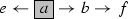. However, $$s_{R}(c,f) \ne 0$$ since there is a path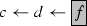with one direction “$$\leftarrow$$” from f to c. Hence, both RWR and SimRank may encounter “zero-similarity” issues.

### 3.5 “Zero-similarity” issue in ASCOS++

Recently, Chen and Giles  proposed a similarity model, ASCOS++,Footnote 1 to address the SimRank issue that “if the length of a path between two nodes is an odd number, this path makes no contribution to the SimRank score”. The issue is a special case of our “zero-similarity” issue. More specifically,  pointed out a sufficient condition for $$s(a,b)=0$$, whereas we give a sufficient and necessary condition for $$s(a,b)=0$$. That is, “the odd-length path between two nodes a and b” provided by  is not the only condition that will lead to $$s(a,b)=0$$. Another condition that “the even-length in-linked paths between nodes a and b whose ‘source’ node is not in the center of the path” will also result in $$s(a,b)=0$$. Therefore, ASCOS++ only partially resolved our “zero-similarity” issue of SimRank. To clarify this, let us look at the ASCOS++ similarity matrix $$\mathbf {S_A}$$ defined by :

\begin{aligned} {[}\mathbf {S_A}]_{i,j} = \left\{ \begin{array}{ll} \tfrac{C}{|\mathcal {I}(i)|} \sum _{x \in \mathcal {I}(i)} {[\mathbf {S_A}]_{x,j}}, &{} i \ne j ; \\ 1, &{} i=j. \end{array} \right. \end{aligned}
(8)

The following theorem shows that ASCOS++ has a RWR-like “zero-similarity” problem.

### Theorem 4

For any two distinct nodes a and b in $${\mathcal {G}}$$, ASCOS++ similarity $$s_A(a,b)$$ defined by Eq. (8) will ignore the contributions of non-unidirectional paths from b to a. As an extreme case, $$s_A(a,b)=0$$ whenever there are no unidirectional paths in $${\mathcal {G}}$$ from b to a.

### Proof

In matrix forms, Eq. (8) can be rewritten as:

\begin{aligned} \mathbf {S_A} = \max \{ C \cdot {\mathbf {Q}} \cdot \mathbf {S_A}, {\mathbf {I}}_n \} = C \cdot {\mathbf {Q}} \cdot \mathbf {S_A} + {\mathbf {D}} \end{aligned}
(9)

where $${\mathbf {D}}={\mathbf {I}}_n - diag(C \cdot {\mathbf {Q}} \cdot \mathbf {S_A})$$ is a diagonal matrix, and $${\mathbf {Q}}$$ is the row-normalized matrix of $${\mathbf {A}}^\mathrm{T}$$.

We rearrange the terms in Eq. (9) and obtain

\begin{aligned} \textstyle \mathbf {S_A} = ( {\mathbf {I}}_n - C \cdot {\mathbf {Q}})^{-1} \cdot {\mathbf {D}} = \sum _{k=0}^{\infty } {C^k \cdot {\mathbf {Q}}^k} \cdot {\mathbf {D}}, \end{aligned}

whose component form is

\begin{aligned} \textstyle [\mathbf {S_A}]_{i,j} = \sum _{k=0}^{\infty } {C^k \cdot {[}{\mathbf {Q}}^k}]_{i,j} \cdot [{\mathbf {D}}]_{j,j}. \end{aligned}
(10)

As $$0\le {{[\mathbf {S_A}]}_{x,y}}\le 1$$ and $$0 \le \sum _{x=1}^{n} {{[{\mathbf {Q}}]}_{i,x}} \le 1$$, we have

\begin{aligned} \textstyle {{[{\mathbf {D}}]}_{j,j}} = 1-C\cdot \sum _{x=1}^{n}{{{[{\mathbf {Q}}]}_{j,x}}\cdot {{{[\mathbf {S_A}]}_{x,j}}}} \ge 1-C >0 \ \ (\forall j) \end{aligned}

In the following, we show that $$[\mathbf {S_A}]_{i,j} \ne 0$$ whenever there exists a unidirectional path from j to i.

(Sufficiency) We first prove that

$$\exists$$ a unidirectional path from j to $$i \Rightarrow [\mathbf {S_A}]_{i,j} \ne 0$$”.

If there exists a unidirectional path from j to i (its length is denoted by $$l_0$$), i.e., $$\underbrace{j\rightarrow \circ \rightarrow \cdots \rightarrow \circ \rightarrow i}_{\text {length } l_0}$$, then it follows from Lemma 1 that

\begin{aligned} {[}({\mathbf {A}}^\mathrm{T})^{l_0}]_{i,j} \ne 0 \ \Leftrightarrow \ {[}{\mathbf {Q}}^{l_0}]_{i,j} > 0 \end{aligned}

because $${\mathbf {Q}}$$ is the row-normalized matrix of $${\mathbf {A}}^\mathrm{T}$$.

As each term $$[{\mathbf {Q}}^l]_{i,j} \ge 0 \ \ (\forall l, \forall i, \forall j)$$ in Eq. (10) and $${{[{\mathbf {D}}]}_{j,j}} \ge 1-C >0 \ \ (\forall j)$$, we have

\begin{aligned} {[}\mathbf {S_A}]_{i,j} \ge \underbrace{C^{l_0}}_{>0} \cdot \underbrace{[{\mathbf {Q}}^{l_0} ]_{i,j}}_{>0} \cdot \underbrace{{[{\mathbf {D}}]}_{j,j}}_{>0} >0. \end{aligned}

(Necessity) We next prove that

$$[\mathbf {S_R}]_{i,j} \ne 0 \Rightarrow \exists$$ a unidirectional path from j to i”.

If $$[\mathbf {S_A}]_{i,j} \ne 0$$, then it follows from Eq. (10) that there exists a term ($$l_0$$-th term) s.t. $$[{\mathbf {Q}}^{l_0}]_{i,j} \cdot [{\mathbf {D}}]_{j,j} > 0$$. Since $${{[{\mathbf {D}}]}_{j,j}} \ge 1-C >0 \ \ (\forall j)$$, it follows that $$[{\mathbf {Q}}^{l_0}]_{i,j} > 0$$.

As $${\mathbf {Q}}$$ is the row-normalized matrix of $${\mathbf {A}}^\mathrm{T}$$, we have

\begin{aligned}{}[{\mathbf {Q}}^{l_0}]_{i,j}> 0 \ \Leftrightarrow \ [({\mathbf {A}}^\mathrm{T})^{l_0}]_{i,j} > 0 \end{aligned}

By Lemma 1, there exists a unidirectional path of length $$l_0$$ from j to i, i.e., $$\underbrace{j\rightarrow \circ \rightarrow \cdots \rightarrow \circ \rightarrow i}_{\text {length } l_0}$$. $$\square$$

The proofs of Theorems 3 and 4 imply that node-pairs of “zero similarities” in both RWR and ASCOS++ models are the same. Indeed, by comparing their power series forms, we notice that RWR and ASCOS++ are almost the same in tallying unidirectional paths except weight assignment for each path.

The probability that the extreme cases of the “zero-similarity” problems for RWR and ASCOS++ stated in Theorems 3 and 4 are often small in practice. This is especially evident for undirected graphs because, for an undirected graph, if the RWR (resp. ASCOS++) similarity $$s(a,b)=0$$, it means there are no connectivity between nodes a and b, i.e., node a and b belong to two different components of a graph. Therefore, the importance of Theorems 3 and 4 is to highlight that, in non-extreme cases where the RWR (resp. ASCOS++) similarity between two nodes is not zero, there are still a number of non-unidirectional paths that can be ignored by the RWR (resp. ASCOS++) model.

Summary In a nutshell, both Jeh and Widom’s SimRank  and Li et al. ’s SimRank  only capture symmetric in-link paths (whose “source” node is in the center), whereas RWR  and ASCOS++  only capture unidirectional paths (whose “source” node is at the right end). All these models have “zero-similarity” problems in digraphs, leading to a biased way of assessing similarity.

## 4 SimRank*: a remedy for SimRank

### 4.1 Geometric series form of SimRank*

As SimRank (resp. RWR) loses asymmetric (resp. non-unidirectional) in-link paths to assess node-pair s(ij), our treatment aims to compensate s(ij) for such a loss, by accommodating asymmetric (resp. non-unidirectional) in-link paths. Precisely, we add the terms $${[{\mathbf {Q}}^{l_1} \cdot {({\mathbf {Q}}^\mathrm{T})}^{l_2}]}_{i,j}$$, $$\forall l_1 \ne l_2$$ (resp. $$\forall l_1 \ne 0$$), with appropriate weights, into the series form of SimRank (resp. RWR) as follows:

### Definition 3

Let $$\hat{{\mathbf {S}}}$$ be a SimRank* similarity matrix. The geometric series form of SimRank* is defined as

\begin{aligned} \hat{{\mathbf {S}}}=(1-C)\cdot \sum _{l=0}^{\infty } {\frac{C^l}{2^l}} \cdot \sum _{\alpha =0}^{l} {l \atopwithdelims ()\alpha } \cdot {\mathbf {Q}}^{\alpha } \cdot {({\mathbf {Q}}^\mathrm{T})}^{l-\alpha }. \end{aligned}
(11)

where $${l \atopwithdelims ()\alpha } \triangleq \frac{l!}{\alpha ! (l-\alpha )!}$$ denotes a binomial coefficient. $$\square$$

To see how the geometric form of SimRank* Eq. (11) is derived and why it resolves the “zero-similarity” problems for SimRank and RWR, we rewrite Eq. (11) as

\begin{aligned}&{[\hat{{\mathbf {S}}}]}_{i,j} = (1-C)\cdot \sum \limits _{l=0}^{\infty } C^l \cdot {[{\hat{{\mathbf {T}}}}_{l}]}_{i,j}\quad \text { with }\nonumber \\&{[{\hat{{\mathbf {T}}}}_{l}]}_{i,j} = \frac{1}{2^l} \cdot \sum _{\alpha =0}^{l} {l \atopwithdelims ()\alpha } \cdot {[{\mathbf {Q}}^{\alpha } \cdot {({\mathbf {Q}}^\mathrm{T})}^{l-\alpha }]}_{i,j} \quad (\forall i, \forall j)\nonumber \\ \end{aligned}
(12)

To avoid ambiguity, in the following, we shall use $$\hat{{\mathbf {S}}}$$ to denote the exact (geometric) SimRank* in Eq. (11).

Comparing Eq. (12) with Li et al. ’s SimRank

\begin{aligned} \textstyle {[{\mathbf {S}}]}_{i,j}=(1-C)\cdot \sum \nolimits _{l=0}^{\infty } C^l \cdot {[{\mathbf {Q}}^l \cdot {({\mathbf {Q}}^\mathrm{T})}^l]}_{i,j} \end{aligned}
(13)

we see that for a fixed l, SimRank* $${\hat{s}}(i,j)$$ uses $$\sum _{\alpha =0}^{l} {l \atopwithdelims ()\alpha } \cdot {[{\mathbf {Q}}^{\alpha } \cdot {({\mathbf {Q}}^\mathrm{T})}^{l-\alpha }]}_{i,j}$$ in $${[{\hat{{\mathbf {T}}}}_{l}]}_{i,j}$$ that captures all in-link paths of length l for node-pair (ij) in a comprehensive way, as opposed to SimRank s(ij) that uses $${[{\mathbf {Q}}^l \cdot {({\mathbf {Q}}^\mathrm{T})}^l]}_{i,j}$$ in Eq. (13) to accommodate only symmetric in-link paths of length 2l for node-pair (ij) in a biased manner. As a result, SimRank* captures all (asymmetric) in-link paths that are ignored by SimRank: (a) in-link paths of odd length; (b) in-link paths of even length whose “source” node is not in the center of the path.

Although RWR and ASCOS++ capture part of in-link paths of odd length that are missed by SimRank, they ignore two types of non-unidirectional in-link paths that can be captured by SimRank*: (a) symmetric ones that are accommodated by SimRank; (b) asymmetric ones whose “source” node is not at the right end.

For instance, given node-pair (ij), Fig. 2 compares all the in-link paths of length $$l \in [1,4]$$ that are captured by Jeh and Widom’s SimRank , Li et al. ’s SimRank , RWR , ASCOS++ , and SimRank*. It can be noticed from ‘SimRank*’ column that only a small number of in-link paths are captured by SimRank (dark gray cells) and RWR/ASCOS++ (light gray cells).

### 4.2 Weighted factors of two types

We next describe two kinds of weighted factors adopted by SimRank* model Eq. (11): (a) length weights$$\{C^l\}_{l=0}^{\infty }$$; and (b) symmetry weights$$\{{l \atopwithdelims ()\alpha }\}_{\alpha =0}^{l}$$.

Intuitively, the length weight$$C^l \ (0<C<1)$$ measures the importance of in-link paths of different lengths. Similar to the original SimRank (Eq. (13)), the outer summation over l in SimRank* (Eq. (12)) is to add up the contributions of in-paths of different length l. The length weight $$C^l$$ aims to reduce the contributions of in-paths of long lengths relative to short ones as $$\{C^l\}_{l\in [0,\infty )}$$ is a deceasing sequence w.r.t. length l.

The symmetry weight uses binomial $${l \atopwithdelims ()\alpha } \ \ (0 \le \alpha \le l)$$ to assess the importance of in-link paths of a fixed length l, with $$\alpha$$ edges in one direction (from the “source” node to one end of the path) and $$l-\alpha$$ edges in the opposite direction, where $$\alpha$$ reflects the symmetry of in-link paths of length l. As depicted in Fig. 2, when $$\alpha = 0 \text { or } l$$, in-link paths become completely asymmetric, reducing to a single direction; when $$\alpha$$ is close to $$\lfloor l/2 \rfloor$$, the “source” node is near the center of in-link paths, being almost symmetric.

To show that the use of binomial $${l \atopwithdelims ()\alpha }$$ is reasonable, in “Appendix B”, we will answer the following questions:

(a) Given a lengthl, why binomial value$${l \atopwithdelims ()\alpha }$$is assigned only to$$l+1$$kinds ofin-link paths?For example, given length$$l=4$$in Fig.2, why ignore the following paths?

\begin{aligned} \rho _1: i\rightarrow \circ \leftarrow \circ \rightarrow \circ \leftarrow j, \quad \rho _2: i\leftarrow \star \rightarrow \circ \leftarrow \diamond \rightarrow j \end{aligned}

(b) Why use binomial value$${l \atopwithdelims ()\alpha }$$, instead of others, to weigh in-link paths?

(c) Why symmetric in-link paths are considered as more important than less symmetric ones, for a given length?

The use of $$(1-C)$$ and $$\frac{1}{2^l}$$ in Eq. (12) is to normalize $${[\hat{{\mathbf {S}}}]}_{i,j}$$ and $${[\hat{{\mathbf {T}}}_{l}]}_{i,j}$$, respectively, into [0, 1]. Specifically, we can verify that $${\Vert {\mathbf {Q}}^{l_1} \cdot {({\mathbf {Q}}^\mathrm{T})}^{l_2} \Vert }_{\max } \le 1 \ \ (\forall l_1, \forall l_2)$$. Thus, (i) $${ \Vert \sum _{\alpha =0}^{l} {l \atopwithdelims ()\alpha } \cdot {{\mathbf {Q}}^{\alpha } \cdot {({\mathbf {Q}}^\mathrm{T})}^{l-\alpha }} \Vert }_{\max } \le \sum _{\alpha =0}^{l} {l \atopwithdelims ()\alpha } = 2^l$$, which implies $${\Vert {\hat{{\mathbf {T}}}}_{l}\Vert }_{\max } \le 1$$. (ii) As $${\Vert \sum _{l=0}^{\infty } C^l \cdot {\hat{{\mathbf {T}}}}_{l}\Vert }_{\max } \le \sum _{l=0}^{\infty } C^l = \frac{1}{1-C}$$, it follows that $${\Vert {{{\mathbf {S}}}}\Vert }_{\max } \le 1$$.

By combining these two kinds of weights, the contribution of any in-link paths for a given node-pair can be easily assessed. For example in Fig. 1,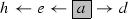has a contribution rate of $$(1-0.8) \cdot {0.8}^3 \frac{1}{2^3} {3 \atopwithdelims ()2} = 0.0384$$ for node-pair (hd). As opposed to SimRank that uses only length weight $$C^l$$, SimRank* considers both $$C^l$$ and symmetry weight $$l \atopwithdelims ()\alpha$$.

### 4.3 Some extensions of SimRank* beyond counting in-link paths only

It is worth mentioning that, in this paper, our proposed SimRank* model mainly focuses on counting in-link paths since our SimRank* follows the SimRank framework that is in-link based. Although SimRank* counts more (asymmetric) in-link paths than SimRank with no compromise in computational time, it should be pointed out that there are some other cases of similar node pairs with zero-similarity values that could not be captured by counting in-link paths only. For example, consider the following path between node a and b:

\begin{aligned} a \leftarrow \circ \rightarrow \circ \rightarrow \circ \leftarrow \circ \rightarrow b \end{aligned}

This path could not be captured by SimRank* since it is not an in-link path. However, we can extend the SimRank* model further by traversing both incoming and outgoing edges, just as the way that Zhao et al.  extended SimRank to P-Rank by taking into account both in- and out-neighboring information. Similar to our Theorem 1, it can be shown that the existing P-Rank model  implies a SimRank-like “zero-similarity” problem, i.e., P-Rank captures only the paths in which every two edges at the symmetric positions of the path have different directions. For example, the following path:

\begin{aligned} a \overset{1}{\leftarrow } \circ \overset{2}{\rightarrow } \circ \overset{3}{\leftarrow } \circ \overset{4}{\rightarrow } \circ \overset{5}{\leftarrow } \circ \overset{6}{\rightarrow } b \end{aligned}

can be captured by P-Rank because (i) at the symmetric position (1, 6), the two edges $$\overset{1}{\leftarrow }$$ and $$\overset{6}{\rightarrow }$$ have different directions; (ii) this also holds for the symmetric position (2, 5) and (3, 4), respectively. However, the path below:

\begin{aligned} a \overset{1}{\leftarrow } \circ \overset{2}{\rightarrow } \circ \overset{3}{\leftarrow } \circ \overset{4}{\rightarrow } \circ \overset{5}{\leftarrow } \circ \overset{6}{\leftarrow } b \end{aligned}

cannot be captured by P-Rank since, at the symmetric position (1, 6), the two edges $$\overset{1}{\leftarrow }$$ and $$\overset{6}{\leftarrow }$$ have the same directions. Fortunately, we can capture this path by extending P-Rank into a new model (namely, P-Rank*), which follows a similar way that we extend SimRank to SimRank*. In our future work, we will formulate the P-Rank* model in detail, and we will show that P-Rank* can count not only in-link paths, but also other newly introduced paths that consist of a mixture of incoming and outgoing edges in any arbitrary positions, without compromising speedup. The P-Rank* model will be more general than SimRank*, but the key idea to extend P-Rank to P-Rank* is similar to the idea that extends SimRank to SimRank*. Thus, in this paper, we mainly focus on the SimRank* model.

It is also worth mentioning that our proposed SimRank* model that determines the similarity by counting in-link paths also can be combined with other structural-context similarity models (e.g., RoleSim  that considers automorphism similarity relationship) to produce a comprehensive similarity measure.

### 4.4 Convergence of SimRank*

As SimRank* in Eq. (11) is an infinite geometric series, it is imperative to study the convergence of this series.

Let us first define thek-th partial sum of Eq. (11) as

\begin{aligned} \hat{{\mathbf {S}}}_k=(1-C)\cdot \sum _{l=0}^{k} {\frac{C^l}{2^l}} \cdot \sum _{\alpha =0}^{l} {l \atopwithdelims ()\alpha } \cdot {\mathbf {Q}}^{\alpha } \cdot {({\mathbf {Q}}^\mathrm{T})}^{l-\alpha }. \end{aligned}
(14)

Using $$\hat{{\mathbf {S}}}_k$$, we next show the convergence of Eq. (11).

### Theorem 5

Let $$\hat{{\mathbf {S}}}$$ and $$\hat{{\mathbf {S}}}_k$$ be defined by Eqs.(11) and (14), respectively. Then, the gap between $$\hat{{\mathbf {S}}}$$ and $$\hat{{\mathbf {S}}}_k$$ is bounded by

\begin{aligned} {\Vert \hat{{\mathbf {S}}} - \hat{{\mathbf {S}}}_k \Vert }_{\max } \le C^{k+1}. \quad (\forall k=0,1,\ldots ) \end{aligned}
(15)

(Please see “Appendix A.3” for the proof of Theorem 5).

### 4.5 Exponential series form of SimRank* variant

In the geometric series form of SimRank* model Eq. (11), Theorem 5 implies that, to guarantee the accuracy $$\epsilon$$, the K-th partial sum $$\hat{{\mathbf {S}}}_K$$ with $$K=\lceil \log _C \epsilon \rceil$$ can be used to approximate the exact solution. However, there is a variant of SimRank* that can use only the $$K'$$-th partial sum with $$K' \le K$$ to guarantee the same $$\epsilon$$:

\begin{aligned} {\hat{{\mathbf {S}}}}'=e^{-C} \cdot \sum _{l=0}^{\infty } {\frac{C^l}{l! }} \cdot \frac{1}{ 2^l} \sum _{\alpha =0}^{l} {l \atopwithdelims ()\alpha } \cdot {\mathbf {Q}}^{\alpha } \cdot {({\mathbf {Q}}^\mathrm{T})}^{l-\alpha }. \end{aligned}
(16)

We call Eq. (16) the exponential series form of SimRank* variant. It differs from Eq. (11) in (i) length weight $$\frac{C^l}{l!}$$ (which is an exponential sequence w.r.t. l) and (ii) its normalized factor $$e^{-C}$$.

The exponential series form of SimRank* is introduced to improve the rate of convergence for similarity computation. To clarify this, we define $${\hat{{\mathbf {S}}}}'_k$$ as the k-th partial sum of $${\hat{{\mathbf {S}}}}'$$ in Eq. (16). Analogous to Theorem 5, one can readily prove

\begin{aligned} {\Vert {\hat{{\mathbf {S}}}}' - {\hat{{\mathbf {S}}}}'_k \Vert }_{\max } \le \tfrac{C^{k+1}}{(k+1)!}. \quad (\forall k=0,1,\ldots ) \end{aligned}
(17)

Comparing Eq. (17) with Eq. (15), we see that for any fixed k, since $$\tfrac{C^{k+1}}{(k+1)!} \le C^{k+1}$$, the convergence rate of $${\hat{{\mathbf {S}}}}'_k$$ is always faster than that of $${\hat{{\mathbf {S}}}}_k$$. Hence, to guarantee the same accuracy, the exponential SimRank* only needs to compute a tiny fraction of the partial sums of the geometric SimRank*.

The choice of length weight $$\tfrac{C^l}{l!}$$ for the exponential SimRank* (Eq. (16)) plays a key role in accelerating convergence. As suggested by the proof of Theorem 5, the bound $$C^{k+1}$$ in Eq. (15) (resp. $$\tfrac{C^{k+1}}{(k+1)!}$$ in Eq. (17)) is actually derived from our choice of length weight $$C^l$$ (resp. $$\tfrac{C^l}{l!}$$) for the geometric (resp. exponential) SimRank*. Thus, there might exist other length weights for speeding up the convergence of SimRank*, as there is no sanctity of the earlier choices of length weight. That is, apart from $$C^l$$ and $$\tfrac{C^l}{l!}$$, other sequence, e.g., $$\tfrac{C^l}{l}$$, that satisfies decreasing monotonicity w.r.t. length l can be regarded as another possible candidate for length weight, since the efficacy of the length weight is to reduce the contributions of in-link paths of long lengths relative to short ones. The reasons why we select $$C^l$$ and $$\tfrac{C^l}{l!}$$, instead of others, are twofold: (i) The normalized factor of length weight should have a simple form, e.g., $$\sum _{l=0}^{\infty } \frac{C^l}{l!}=e^C$$. (ii) Once selected, the length weight should enable the series form of SimRank* to be simplified into a very elegant form, e.g., using $$\frac{C^l}{l!}$$ allows Eq. (16) being simplified, as will be seen in Eq. (20), into a neat closed form. In contrast, $$\tfrac{C^l}{l}$$ is not a preferred length weight as its series version may not be simplified into a neat recursive (or closed) form, though the form $$\sum _{l=0}^{\infty } \frac{C^l}{l}= \ln {\tfrac{1}{(1-C)}}$$ is simple for normalized factor.

## 5 Recursive and closed forms of SimRank*

A brute-force way of computing the first k-th partial sums of Eq. (11) requires $$O(k\cdot l^2 \cdot n^3)$$ time, involving $$l^2$$ matrix multiplications in the inner summation for each fixed l in the outer summation, which seems much more expensive than SimRank. In this section, we propose two simple representations of SimRank* (i.e., the recursive form of geometric SimRank*, and the closed form of exponential SimRank*).

### 5.1 Recursive form of geometric SimRank*

We first show the recursive form of the geometric SimRank* series in Eq. (11).

### Theorem 6

The SimRank* geometric series $$\hat{{\mathbf {S}}}$$ in Eq. (11) takes the following elegant recursive form:

\begin{aligned} \hat{{\mathbf {S}}} = \tfrac{C}{2} \cdot ({\mathbf {Q}}\cdot \hat{{\mathbf {S}}} + \hat{{\mathbf {S}}}\cdot {\mathbf {Q}}^\mathrm{T} ) + (1-C) \cdot {\mathbf {I}}_n. \end{aligned}
(18)

(Please see “Appendix A.4” for the proof of Theorem 6).

Theorem 6 provides a time-efficient iterative algorithm to compute SimRank* matrix $$\hat{{\mathbf {S}}}_k$$, with its accuracy guaranteed by Theorem 5. The complexity of this iterative method is O(Knm) time and $$O(n^2)$$ memory. Please refer to “Appendix C” for a detailed analysis.

The $$O(n^2)$$ memory of Eq. (18) is the main barrier that hinders the scalability of SimRank* on large graphs. In Sect. 7, we will provide a scalable algorithm, named ss-gSR*, that will substantially reduce the memory from quadratic to linear, without any loss of accuracy.

Compared with SimRank that follows a simple idea that “two distinct nodes are similar if their in-neighbors are similar”, Theorem 6 implies a simple SimRank-like concept to describe the basic philosophy of SimRank*, i.e., “two distinct nodes are similar if either node and the in-neighbors of the other node are similar.” Indeed, for two distinct nodes a and b, when their in-neighbors are not empty, this simple idea of SimRank* is observed by rewriting Eq. (18) into the following component form:

\begin{aligned} {\hat{s}}(a,b) =\frac{C}{2} \Bigg ( \underbrace{\tfrac{\sum \nolimits _{y \in {\mathcal {I}}\left( b \right) }{{\hat{s}}(a,y)}}{\left| {\mathcal {I}}(b) \right| }}_{\textsf {Part 1}} + \underbrace{\tfrac{\sum \nolimits _{x \in {\mathcal {I}}\left( a \right) }{{\hat{s}}(x,b)}}{\left| {\mathcal {I}}(a) \right| }}_{\textsf {Part 2}} \Bigg ) \quad (a \ne b)\nonumber \\ \end{aligned}
(19)

where SimRank* similarity $${\hat{s}}(a,b)$$ consists of two parts: (i) Part 1 is the average similarity between node a and node b’s in-neighbors; (ii) Part 2 is the average similarity between node b and node a’s in-neighbors.

### 5.2 Closed form of exponential SimRank*

Having converted the series form of geometric SimRank* into a simple recursive form, we next present the closed form of exponential SimRank* in Eq. (16).

### Theorem 7

The exponential series form of SimRank* in Eq. (16) neatly takes the following closed form:

\begin{aligned} {\hat{{\mathbf {S}}}}' = {{e}^{-C}}\cdot {{e}^{\tfrac{C}{2}{\mathbf {Q}}}}\cdot {{e}^{\tfrac{C}{2}{{{\mathbf {Q}}}^{T}}}}, \end{aligned}
(20)

where exponential $${{e}^{{\mathbf {X}}}}\triangleq {\mathbf {I}}+{\mathbf {X}}+\frac{{{{\mathbf {X}}}^{2}}}{2!} +\cdots =\sum _{k=0}^{\infty }{\frac{{{{\mathbf {X}}}^{k}}}{k!}}$$ for a square matrix $${\mathbf {X}}$$.

(Please see “Appendix A.5” for the proof of Theorem 7).

The utility of Theorem 7 will be shown in Sect. 6.4 for optimizing the exponential SimRank* computation.

## 6 Accelerate SimRank* computation

To accelerate SimRank* iterations in Eq. (50), the conventional optimization techniques  for SimRank cannot be effectively applied to SimRank*. Lizorkin et al.  proposed “partial sums memoization” to optimize SimRank computation. To show why it does not work for SimRank*, let us compare the component forms of SimRank and SimRank* in Eqs.(21) and (22), respectively:

\begin{aligned}&s_{k+1}( a,b ) =\tfrac{C}{| {\mathcal {I}}( a ) | | {\mathcal {I}}( b ) |}\sum \limits _{x \in {\mathcal {I}}(a) } \overbrace{\sum \limits _{y \in {\mathcal {I}}( b ) }{s_{k}( x, y )}}^{= \text {Partial}_{{{\mathcal {I}}}(b)}^{s_k}(x)}. \end{aligned}
(21)
\begin{aligned}&\quad \times {\hat{s}}_{k+1}(a,b) \nonumber \\&\quad =\tfrac{C}{2 \left| {\mathcal {I}}(b) \right| }\underbrace{\sum \limits _{y \in {\mathcal {I}}\left( b \right) }{{\hat{s}}_{k}(a,y)}}_{{= \text {Partial}_{{{\mathcal {I}}}(b)}^{{\hat{s}}_k}(a)}} + \tfrac{C}{2 \left| {\mathcal {I}}(a) \right| }{\sum \limits _{x \in {\mathcal {I}}\left( a \right) }{{\hat{s}}_{k}(x,b)}}. \end{aligned}
(22)

For SimRank, if $${{\mathcal {I}}}(a)$$ and $${{\mathcal {I}}}(\star )$$ have some node, say i, in common, then the partial sum $$\text {Partial}_{{{\mathcal {I}}}(b)}^{s_k}(i)$$ in Eq. (21), once memoized, can be reused in both $${{\hat{s}}}_{k+1}(a,b)$$ and $${{\hat{s}}}_{k+1}(\star ,b)$$ computation. In contrast, for SimRank*, regardless of $${{\mathcal {I}}}(a) \cap {{\mathcal {I}}}(\star ) \ne \varnothing$$, the partial sum $$\text {Partial}_{{{\mathcal {I}}}(b)}^{{\hat{s}}_k}(a)$$ in Eq. (22) for computing $${{\hat{s}}}_{k+1}(a,b)$$, if memoized, has no chance to be reused again in computing other similarities $${{\hat{s}}}_{k+1}(\star , b)$$, where $$\star$$ is any node in $${\mathcal {G}}$$ except a.

### 6.1 Fine-grained memoization

Instead of memoizing the results of $$\sum _{y \in {\mathcal {I}}\left( b \right) }{{\hat{s}}_{k}(a,y)}$$ over the whole set $${{\mathcal {I}}}(b)$$ in Eq. (22), we use fine-grained memoization for optimizing SimRank* by memoizing a partial sum over a subset as follows:

\begin{aligned} \text {Partial}_{{\varDelta }}^{{\hat{s}}_k}(a) \triangleq \sum _{y \in {\varDelta } }{{\hat{s}}_{k}(a,y)} \text { with } {\varDelta } \subseteq {{\mathcal {I}}}(\star ). \end{aligned}

Our observation is that there may be duplicate additions among $$\sum _{y \in {\mathcal {I}}\left( \star \right) }{{\hat{s}}_{k}(a,y)}$$ over different in-neighbor sets $${{\mathcal {I}}}(\star )$$. Thus, once memoized, the result of $$\text {Partial}_{{\varDelta }}^{{\hat{s}}_k}(a)$$ can be shared among many sums $$\sum _{y \in {\mathcal {I}}\left( \star \right) }{{\hat{s}}_{k}(a,y)}$$ for computing $${{\hat{s}}}_{k+1}(a,\star )$$. As an example in Fig. 1, $${{\mathcal {I}}}(h)$$ and $${{\mathcal {I}}}(i)$$ have three nodes $$\{e,j,k\}$$ in common, and thus, once memoized, the resulting fine-grained partial sum $$\text {Partial}_{\{e,j,k\}}^{{\hat{s}}_k}(a)$$ can be shared between $$\sum _{y \in {{\mathcal {I}}}(h)}{{\hat{s}}_{k}(a,y)}$$ and $$\sum _{y \in {{\mathcal {I}}}(i)}{{\hat{s}}_{k}(a,y)}$$ for computing both $${{\hat{s}}}_{k+1}(a,h)$$ and $${{\hat{s}}}_{k+1}(a,i)$$ via Eq. (22), for any fixed a. However, it seems difficult to find perfect fine-grained subsets $${\varDelta } \subseteq {{\mathcal {I}}}(\star )$$ for maximal computation sharing, since there may be many arbitrarily overlapped in-neighbor sets in a graph. To overcome this difficulty, we will employ efficient techniques of bipartite graph compression via edge concentration for finding such fine-grained subsets.

### Definition 4

An induced bipartite graph (bigraph) from a given graph $${{\mathcal {G}}}=({{\mathcal {V}}},{{\mathcal {E}}})$$ is a bipartite graph $$\tilde{{\mathcal {G}}}=({{\mathcal {T}}}\cup {{\mathcal {B}}},\tilde{{{\mathcal {E}}}})$$, such that its two disjoint node sets $${{\mathcal {T}}}=\{x\in {{\mathcal {V}}} \ | \ {{\mathcal {O}}}(x) \ne \varnothing \}$$, $${{\mathcal {B}}}=\{x \in {{\mathcal {V}}} \ | \ {{\mathcal {I}}}(x) \ne \varnothing \}$$,Footnote 2 and for each $$u \in {{\mathcal {T}}}$$ and $$v \in {{\mathcal {B}}}$$, $$(u,v) \in \tilde{{{\mathcal {E}}}}$$ if and only if there is an edge from u to v in $${\mathcal {G}}$$.

Intuitively, an induced bigraph $$\tilde{{\mathcal {G}}}=({{\mathcal {T}}}\cup {{\mathcal {B}}},\tilde{{{\mathcal {E}}}})$$ visualizes the neighborhood structure of $${\mathcal {G}}$$ from a different perspective. For any $$x \in {{\mathcal {B}}}$$, the nodes in $${\mathcal {T}}$$ that are connected with x correspond to the in-neighbors of x in $${\mathcal {G}}$$. Note that when node x has both in- and out-neighbors in $${\mathcal {G}}$$, label x that appears in both $${{\mathcal {T}}}$$ and $${{\mathcal {B}}}$$ will be regarded as two distinct nodes despite the same label. To avoid ambiguity, we shall use $$x \in {{\mathcal {T}}}$$ and $$x \in {{\mathcal {B}}}$$ to distinguish them. Each directed edge in $${\mathcal {G}}$$ is mapped to one edge in $$\tilde{{\mathcal {G}}}$$, and thus, $$|{{\mathcal {E}}}|=|\tilde{{{\mathcal {E}}}}|$$. For instance, the left part of Fig. 3 shows the induced bigraph $$\tilde{{\mathcal {G}}}$$ from $${\mathcal {G}}$$ of Fig. 1. From $$\tilde{{\mathcal {G}}}$$, we can clearly see that b and d in $${\mathcal {B}}$$ are both connected with a in $${\mathcal {T}}$$, meaning that, in $${\mathcal {G}}$$, b and d both have an in-neighbor a.

### 6.3 Biclique compression via edge concentration

Based on the induced bigraph $$\tilde{{\mathcal {G}}}$$, we next introduce the notion of bipartite cliques (bicliques).

### Definition 5

Given an induced bigraph $$\tilde{{\mathcal {G}}}=({{\mathcal {T}}}\cup {{\mathcal {B}}},\tilde{{{\mathcal {E}}}})$$, a pair of two disjoint subsets $${{\mathcal {X}}} \subseteq {{\mathcal {T}}}$$ and $${{\mathcal {Y}}} \subseteq {{\mathcal {B}}}$$ is called a biclique if $$(x,y) \in \tilde{{{\mathcal {E}}}}$$ for all $$x \in {{\mathcal {X}}}$$ and $$y \in {{\mathcal {Y}}}$$.

Intuitively, a biclique $$({{\mathcal {X}}}, {{\mathcal {Y}}})$$ is a complete bipartite subgraph of $$\tilde{{\mathcal {G}}}$$, which has $$|{{\mathcal {X}}}|+|{{\mathcal {Y}}}|$$ nodes and $$|{{\mathcal {X}}}| \times |{{\mathcal {Y}}}|$$ edges. Each biclique $$({{\mathcal {X}}}, {{\mathcal {Y}}})$$ in $$\tilde{{\mathcal {G}}}$$ implies that, in $${\mathcal {G}}$$, all nodes $$y \in {{\mathcal {Y}}}$$ have the common in-neighbor set $${{\mathcal {X}}}$$. For example, there are two bicliques $$(\{b,d\},\{c,g,i\})$$ in dashed line, and $$(\{e,j,k\},\{h,i\})$$ in dotted line in Fig. 3. Biclique $$(\{b,d\},\{c,g,i\})$$ in $$\tilde{{\mathcal {G}}}$$ implies that in $${\mathcal {G}}$$, nodes cgi have two in-neighbors $$\{b,d\}$$ in common.

Bicliques are introduced to compress bigraph $$\tilde{{\mathcal {G}}}$$ for optimizing SimRank* computation. In “Appendix D.1”, we present the main idea of our bigraph compression techniques. Then, we propose an algorithm, memo-gSR*, for computing all-pairs SimRank* quickly, by using fine-grained memoization (“Appendix D.2”). The correctness and complexity of memo-gSR* are shown in “Appendix D.3”, which requires $$O(K n {\tilde{m}})$$ time and $$O(n^2)$$ memory, followed by a running example in “Appendix D.4”.

To scale memo-gSR* on large graphs, in Sect. 7 we will propose a memory-efficient algorithm, ss-gSR*.

### 6.4 Exponential SimRank* optimization

The aforementioned optimization methods for (geometric) SimRank* computation can be readily extended to exponential SimRank* variant. Please refer to “Appendix D.5” for the optimization techniques generalized to speed up the exponential SimRank* search.

## 7 Linearize SimRank* memory

In Sect. 6, our optimization techniques focus on speeding up the computation of SimRank*, which is based on the following iterative model to evaluate $${\hat{{\mathbf {S}}}}_{k}$$:

\begin{aligned} \left\{ \begin{array}{ll} {\hat{{\mathbf {S}}}}_{0} = (1-C) \cdot {\mathbf {I}}_n, \\ {\hat{{\mathbf {S}}}}_{k} = \frac{C}{2} ({\mathbf {Q}}\cdot {\hat{{\mathbf {S}}}}_{k-1} + {\hat{{\mathbf {S}}}}_{k-1} \cdot {\mathbf {Q}}^\mathrm{T} ) + (1-C) \cdot {\mathbf {I}}_n. \end{array} \right. \end{aligned}
(23)

However, the memory space of the above iteration entails $$O(n^2)$$. This is because, for each iteration of Eq. (23), even if we want to compute a single entry of $${\hat{{\mathbf {S}}}}_{k}$$ at the k-th iteration, all $$(n^2)$$ pairs of SimRank* scores $${\hat{{\mathbf {S}}}}_{k-1}$$ at the previous iteration need to be prepared in advance, as pictorially depicted in Fig. 4. This would hinder the scalability of SimRank* on large graphs.

To resolve this problem, in this section, we propose a memory-efficient version of SimRank*, which linearizes the memory space of Eq. (14) without loss of accuracy. Let us now recall the k-th partial sum of the SimRank* power series form in Eq. (14):

\begin{aligned} \hat{{\mathbf {S}}}_k=(1-C)\cdot \sum _{l=0}^{k} {\frac{C^l}{2^l}} \cdot \sum _{\alpha =0}^{l} {l \atopwithdelims ()\alpha } \cdot {\mathbf {Q}}^{\alpha } \cdot {({\mathbf {Q}}^\mathrm{T})}^{l-\alpha }. \end{aligned}
(24)

From the proof of Theorem 6 in Sect. 5.1, we discern that the k-th partial sum of the SimRank* power series in Eq. (24) produces exactly the same results as the k-th iterative SimRank* model in Eq. (23). Since the right-hand side of Eq. (24) depends only on $${\mathbf {Q}}$$ and C, we can compute $$\hat{{\mathbf {S}}}_k$$ in a column-by-column fashion, which requires only linear memory. However, a key challenge is that there are many unnecessary duplicate computations that will greatly increase the overheads. Precisely, let $${\mathbf {e}}_q$$ be an $$n\times 1$$ unit vector: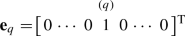If we multiply $${\mathbf {e}}_q \ (q=1,\ldots ,n)$$ on both sides of Eq. (24), it will produce

\begin{aligned}{}[\hat{{\mathbf {S}}}_k]_{*,q}=(1-C) \sum _{l=0}^{k} {\frac{C^l}{2^l}} \sum _{\alpha =0}^{l} {l \atopwithdelims ()\alpha } {\mathbf {Q}}^{\alpha } {({\mathbf {Q}}^\mathrm{T})}^{l-\alpha } {\mathbf {e}}_q. \end{aligned}
(25)

We notice that, if the matrix-vector multiplications in the right-hand side of Eq. (25) are carried out as below:

\begin{aligned}&{{{\mathbf {Q}}}^{\alpha }}{{({{{\mathbf {Q}}}^{T}})}^{l-\alpha }}{{{\mathbf {e}}}_{q}} \\&= \underbrace{\Big ( {\mathbf {Q}}\cdot \cdots \cdot \Big ( {\mathbf {Q}}\cdot \Big ( {\mathbf {Q}}}_{\alpha \text { times}} \cdot \underbrace{\Big ({{{\mathbf {Q}}}^{T}}\big ( \cdot \cdots \cdot \big ( {{{\mathbf {Q}}}^{T}}\cdot \big ( {{{\mathbf {Q}}}^{T}}}_{(l-\alpha ) \text { times}} \cdot {{{\mathbf {e}}}_{q}} \big ) \big ) \big ) \Big ) \Big ) \Big ) \Big ) \end{aligned}

it requires only O(m) memory to compute Eq. (25) (which is dominated by matrix-vector multiplications), but the computational time is prohibitively expensive. Indeed, due to double summations in Eq. (25), given l and $$\alpha$$, it requires $$\alpha +(l-\alpha )$$ matrix-vector multiplications to compute $${{{\mathbf {Q}}}^{\alpha }}{{({{{\mathbf {Q}}}^{T}})}^{l-\alpha }}{{{\mathbf {e}}}_{q}}$$. Therefore, the total number of matrix-vector multiplications required for Eq. (25) is

\begin{aligned} \sum _{l=0}^k \sum _{\alpha =0}^{l} \big (\alpha +(l-\alpha ) \big ) = \sum _{l=0}^k {(l+1) l} = \frac{k(k+1)(k+2)}{3} \end{aligned}

which is rather costly. However, we observe that there are many duplicate computations across the double summations in Eq. (25). For example, let us consider the two cases when $$l=4, \alpha =1$$ and $$l=2, \alpha =0$$, respectively. There are overlapping matrix-vector multiplications between $${{{\mathbf {Q}}}^{1}}{{({{{\mathbf {Q}}}^{T}})}^{3}}{{{\mathbf {e}}}_{q}}$$ and $${{{\mathbf {Q}}}^{0 }}{{({{{\mathbf {Q}}}^{T}})}^{2}}{{{\mathbf {e}}}_{q}}$$, as shown below:Thus, it is imperative to devise an efficient method that can remove duplicate computations by reusing overlapping parts for subsequent repeated multiplications.

### 7.1 Single-source geometric SimRank*

To efficiently compute a single column of the SimRank* matrix $$\hat{{\mathbf {S}}}_k$$, we first focus on geometric SimRank* search, and propose an efficient method that requires only linear memory while minimizing duplicate computations without any loss of accuracy.

### Theorem 8

(Single-Source Geometric SimRank*) Given query q, the single-source geometric SimRank* between all nodes and q at the k-th iteration of Eq. (23), denoted as $${{[{{{\hat{\mathbf {S}}}}_{k}}]}_{*,q}}$$, can be iteratively computed as

\begin{aligned} {{[{{{\hat{\mathbf {S}}}}_{k}}]}_{*,q}}=(1-C)\cdot {{{\mathbf {u}}}_{k}} \end{aligned}
(26)

where the vector $${{{\mathbf {u}}}_{k}}$$ is iteratively derived by

\begin{aligned} \left\{ \begin{array}{l} {{{\mathbf {u}}}_{0}}={\mathbf {m}}_{k+1}^{(k)} \\ {{{\mathbf {u}}}_{i}}={\mathbf {m}}_{k+1}^{(k-i)}+\tfrac{C}{2}\cdot {\mathbf {Q}}\cdot {{{\mathbf {u}}}_{i-1}} \quad (i=1,2,\ldots ,k) \\ \end{array} \right. \end{aligned}
(27)

and $${\mathbf {m}}_{k+1}^{(0)},{\mathbf {m}}_{k+1}^{(1)},\ldots ,{\mathbf {m}}_{k+1}^{(k)}$$ are iteratively obtained by

\begin{aligned} \left\{ \begin{array}{ll} {\mathbf {m}}_{i}^{(-1)}={{{\mathbf {e}}}_{q}} &{} (i=0,1,2,\ldots ,k) \\ {\mathbf {m}}_{i}^{(i)}={\mathbf {0}} &{} (i=0,1,2,\ldots ,k) \\ {\mathbf {m}}_{i}^{(j)}=\tfrac{C}{2}\cdot {{{\mathbf {Q}}}^{T}}\cdot {\mathbf {m}}_{i-1}^{(j)} \qquad &{} (i=1,2,\ldots ,k+1; \\ \quad \qquad {} +{\mathbf {m}}_{i-1}^{(j-1)} &{} \ j=0,1,\ldots ,i-1) \end{array} \right. \end{aligned}
(28)

Before proving Theorem 8, we first give an example to illustrate the application of this theorem to compute single-source SimRank* efficiently.

### Example 3

Recall the graph in Fig. 1. Given query node e, the decay factor $$C=0.6$$, and the number of iterations $$k=3$$, the single-source geometric SimRank* $${{[{{{\hat{\mathbf {S}}}}_{k}}]}_{*,e}}$$ can be computed via Theorem 8 as follows:

First, according to Eq. (28), we iteratively compute the auxiliary vectors $${\mathbf {m}}_{4}^{(0)},{\mathbf {m}}_{4}^{(1)}, {\mathbf {m}}_{4}^{(2)} ,{\mathbf {m}}_{4}^{(3)}$$ as follows:

i

j

$${\mathbf {m}}_{i}^{(j)}$$

1

0

$${\mathbf {m}}_{1}^{(0)} =\tfrac{C}{2} {{{\mathbf {Q}}}^{T}} \overbrace{{\mathbf {m}}_{0}^{(0)}}^{={\mathbf {0}}}+\overbrace{{\mathbf {m}}_{0}^{(-1)}}^{={{{\mathbf {e}}}_{e}}}$$ $$={{[0 , 0, 0, 0, 1, 0, 0, 0, 0, 0, 0]}^{T}}$$

2

0

$${\mathbf {m}}_{2}^{(0)} =\tfrac{C}{2} {{{\mathbf {Q}}}^{T}} {{\mathbf {m}}_{1}^{(0)}}+\overbrace{{\mathbf {m}}_{1}^{(-1)}}^{={{{\mathbf {e}}}_{e}}}$$ $$= [.3, 0, 0, 0, 1, 0, 0, 0, 0, 0, 0]^\mathrm{T}$$

1

$${\mathbf {m}}_{2}^{(1)} =\tfrac{C}{2} {{{\mathbf {Q}}}^{T}} \underbrace{{\mathbf {m}}_{1}^{(1)}}_{={\mathbf {0}}}+{{\mathbf {m}}_{1}^{(0)}}$$ $$={{[0, 0, 0, 0, 1, 0, 0, 0, 0, 0, 0]}^\mathrm{T}}$$

3

0

$${\mathbf {m}}_{3}^{(0)} =\tfrac{C}{2} {{{\mathbf {Q}}}^{T}} {\mathbf {m}}_{2}^{(0)}+\overbrace{{\mathbf {m}}_{2}^{(-1)}}^{={{{\mathbf {e}}}_{e}}}$$ $$=[.3, 0, 0, 0, 1, 0, 0, 0, 0, 0, 0]^\mathrm{T}$$

1

$${\mathbf {m}}_{3}^{(1)} =\tfrac{C}{2} {{{\mathbf {Q}}}^{T}} {\mathbf {m}}_{2}^{(1)}+{\mathbf {m}}_{2}^{(0)}$$ $$=[.6, 0, 0, 0, 1, 0, 0, 0, 0, 0, 0]^\mathrm{T}$$

2

$${\mathbf {m}}_{3}^{(2)} =\tfrac{C}{2} {{{\mathbf {Q}}}^{T}} \underbrace{{\mathbf {m}}_{2}^{(2)}}_{={\mathbf {0}}}+{{\mathbf {m}}_{2}^{(1)}}$$ $$=[0, 0, 0, 0, 1, 0, 0, 0, 0, 0, 0]^\mathrm{T}$$

4

0

$${\mathbf {m}}_{4}^{(0)} =\tfrac{C}{2} {{{\mathbf {Q}}}^{T}} {\mathbf {m}}_{3}^{(0)}+\overbrace{{\mathbf {m}}_{3}^{(-1)}}^{={{{\mathbf {e}}}_{e}}}$$ $$=[.3, 0, 0, 0, 1, 0, 0, 0, 0, 0, 0]^\mathrm{T}$$

1

$${\mathbf {m}}_{4}^{(1)} =\tfrac{C}{2} {{{\mathbf {Q}}}^{T}} {\mathbf {m}}_{3}^{(1)}+{{\mathbf {m}}_{3}^{(0)}}$$ $$=[.6, 0, 0, 0, 1, 0, 0, 0, 0, 0, 0]^\mathrm{T}$$

2

$${\mathbf {m}}_{4}^{(2)} =\tfrac{C}{2} {{{\mathbf {Q}}}^{T}} {\mathbf {m}}_{3}^{(2)}+{\mathbf {m}}_{3}^{(1)}$$ $$=[.9, 0, 0, 0, 1, 0, 0, 0, 0, 0, 0]^\mathrm{T}$$

3

$${\mathbf {m}}_{4}^{(3)} =\tfrac{C}{2} {{{\mathbf {Q}}}^{T}} \underbrace{{\mathbf {m}}_{3}^{(3)}}_{={\mathbf {0}}}+{\mathbf {m}}_{3}^{(2)}$$ $$={{[0, 0, 0, 0, 1, 0, 0, 0, 0, 0, 0]}^\mathrm{T}}$$

Next, based on Eq. (26), we iteratively compute the vector $${\mathbf {u}}_3$$ from $${\mathbf {m}}_{4}^{(0)},{\mathbf {m}}_{4}^{(1)}, {\mathbf {m}}_{4}^{(2)} ,{\mathbf {m}}_{4}^{(3)}$$ as follows:

i

$${\mathbf {u}}_{i}$$

0

$${\mathbf {u}}_{0} = {\mathbf {m}}_{4}^{(3)}$$ $$={{[0, 0, 0, 0, 1, 0, 0, 0, 0, 0, 0]}^\mathrm{T}}$$

1

$${{{\mathbf {u}}}_{1}}={\mathbf {m}}_{4}^{(2)}+\tfrac{C}{2} {\mathbf {Q}} {{{\mathbf {u}}}_{0}}$$ $$=[.9, 0, 0, 0, 1, 0, 0, .3, .1]^\mathrm{T}$$

2

$${{{\mathbf {u}}}_{2}}={\mathbf {m}}_{4}^{(1)}+\tfrac{C}{2} {\mathbf {Q}} {{{\mathbf {u}}}_{1}}$$ $$=[.6, .27, 0, .135, 1.27, 0, 0, .3, .1]^\mathrm{T}$$

3

$${{{\mathbf {u}}}_{3}}={\mathbf {m}}_{4}^{(0)}+\tfrac{C}{2} {\mathbf {Q}} {{{\mathbf {u}}}_{2}}$$ $$=[.3, .18, .061, .09, 1.18, .081, .061, .381, .168]^\mathrm{T}$$

Finally, $${{[{{{\hat{\mathbf {S}}}}_{3}}]}_{*,e}}$$ can be obtained from $${{{\mathbf {u}}}_{3}}$$ via Eq. (26):

\begin{aligned}&{{[{{\hat{\mathbf {S}}}_{3}}]}_{*,e}} =(1-C)\cdot {{{\mathbf {u}}}_{3}} = 0.4 \cdot {{{\mathbf {u}}}_{3}} \\&\quad = \big [.12, .072, .0243, .036, .472, .0324, .0243, .1524, .067\big ]^\mathrm{T} \ \ \end{aligned}

$$\square$$

Theorem 8 efficiently assesses single-source SimRank* by merging duplicate matrix-vector computations, which is due to our novel iterative model Eq. (28) that employs a Pascal’s triangle pattern. Pictorially, Fig. 5 depicts how Eq. (28) iteratively obtains $${\mathbf {m}}_{k+1}^{(0)},{\mathbf {m}}_{k+1}^{(1)}, \ldots , {\mathbf {m}}_{k+1}^{(k)}$$ (in last row) from scratch using a Pascal’s triangle style. To generate the Pascal’s triangle in Fig. 5, we start to write the first row with two elements $${\mathbf {m}}_0^{(-1)}$$ and $${\mathbf {m}}_0^{(0)}$$, which are initialized to $${\mathbf {e}}_q$$ and $${\mathbf {0}}$$, respectively. Then, each new row $$i \ (i=1,2,\ldots ,k+1)$$ is generated as follows: (a) Each new row i starts with $${\mathbf {m}}_i^{(-1)}$$ initialized to $${\mathbf {e}}_q$$, and ends with $${\mathbf {m}}_i^{(i)}$$ initialized to $${\mathbf {0}}$$. (b) The remaining elements $${\mathbf {m}}_i^{(j)} \ (j=1, 2, \ldots ,i-1)$$ in each new row i are derived from two elements $${\mathbf {m}}_{i-1}^{(j)}$$ and $${\mathbf {m}}_{i-1}^{(j-1)}$$ in the row above which lie above and above-left. Thus, every three elements $${\mathbf {m}}_i^{(j)}, {\mathbf {m}}_{i-1}^{(j)}, {\mathbf {m}}_{i-1}^{(j-1)}$$ form a Pascal’s triangle pattern, which means that $${\mathbf {m}}_i^{(j)}$$ is derived from pre-multiplying $${\mathbf {m}}_{i-1}^{(j)}$$ by $$(\tfrac{C}{2} {\mathbf {Q}}^\mathrm{T})$$ plus $${\mathbf {m}}_{i-1}^{(j-1)}$$. For instance, the red Pascal’s triangle pattern in Fig. 5 indicates that $${\mathbf {m}}_3^{(1)}$$ is obtained by pre-multiplying $${\mathbf {m}}_2^{(1)}$$ by $$(\tfrac{C}{2} {\mathbf {Q}}^\mathrm{T})$$ plus $${\mathbf {m}}_{2}^{(0)}$$, i.e., $${\mathbf {m}}_3^{(1)} \leftarrow (\tfrac{C}{2} {\mathbf {Q}}^\mathrm{T}) \cdot {\mathbf {m}}_3^{(1)} + {\mathbf {m}}_{2}^{(0)}$$.

The main advantages of Theorem 8 are fourfold:

1. 1.

It provides a memory-efficient iterative model that allows SimRank* retrieval scaling well on large graphs, without compromising accuracy and with no need to store all $$(n^2)$$ pairs SimRank* scores $$\hat{{\mathbf {S}}}_{k-1}$$ at the previous iteration of Eq. (23). As opposed to the $$O(n^2)$$ memory of the conventional iterative model Eq. (23), our new iterative model in Theorem 8 requires only $$O(kn+m)$$ memory, which is dominated by matrix-vector multiplications $${\mathbf {Q}}\cdot {{{\mathbf {u}}}_{i-1}}$$ in Eq. (27) and $${{{\mathbf {Q}}}^{T}}\cdot {\mathbf {m}}_{i-1}^{(j)}$$ in Eq. (28).

2. 2.

Compared with the straightforward right-to-left association in Eq. (25) that requires $$\frac{k(k+1)(k+2)}{3}$$ matrix-vector multiplications, our novel iterative model in Theorem 8 utilizes a Pascal’s triangle fashion to evaluate $$\{{\mathbf {m}}_{j}^{(i)}\}$$ that effectively eliminates duplicate multiplications and significantly reduces the number of matrix-vector multiplications to

\begin{aligned} \underbrace{\Big ( \sum _{i=1}^{k} 1 \Big )}_{\text {Eq.}(27)} + \underbrace{\Big ( \sum _{i=1}^{k+1} \sum _{j=0}^{i-1} 1 \Big )}_{\text {Eq.}(28)} = k + \frac{(k+1)(k+2)}{2} \end{aligned}
3. 3.

Theorem 8 implies an efficient parallel algorithm for all-pairs SimRank* search. Indeed, the computation of all-pairs SimRank* $$\hat{{\mathbf {S}}}$$ can be broken into n columns $$[\hat{{\mathbf {S}}}]_{*,q} \ (q=1,\ldots ,n)$$ of single-source SimRank* search, where each column can be computed concurrently on different processors via Theorem 8. In contrast, the previous iterative model Eq. (23) to compute all-pairs SimRank* is not parallelizable.

4. 4.

The iterative model in Theorem 8 is query-dependent, which provides an on-demand retrieving strategy for SimRank*. That is, SimRank* scores can be retrieved on an as-needed basis by Theorem 8. In comparison, the previous model Eq. (23) always outputs all-pairs scores even if only a fraction of scores are requested.

Based on Theorem 8, we provide a memory-efficient algorithm, ss-gSR*, for single-source geometric SimRank*. We analyze its complexity and correctness below:

### Theorem 9

(Complexity) Given a graph $${\mathcal {G}}$$, a query q, and the number of iterations K, ss-gSR* requires $$O(Kn+m)$$ memory and $$O(K^2m)$$ time to iteratively compute single-source geometric SimRank* scores $${{[{{\hat{\mathbf {S}}}_{K}}]}_{\star ,q}}$$.

(Please see “Appendix A.6” for the proof of Theorem 9).

It is worth mentioning that our edge concentration approach in Sect. 6 can be integrated with ss-gSR* to enable a further speedup of single-source SimRank* retrieval. We just need to replace $${\mathbf {Q}}$$ of $${\mathcal {G}}$$ with the new backward transition matrix of the compressed graph of $${\mathcal {G}}$$ in Algorithm 1. Then, the total time of ss-gSR* becomes $$O(K^2{\tilde{m}} + {\tilde{m}} \log (2n))$$ time, where $${\tilde{m}}$$ is the number of edges in the compressed graph, and $$O({\tilde{m}} \log (2n))$$ is the time required for graph compression.

Correctness To show that the results $$\hat{s}_{K}(\star ,q)$$ output by ss-gSR* are correct, let us first propose the following two lemmas, which will be used to prove Theorem 8.

### Lemma 2

For each iteration $$i=0,1,\ldots ,k$$, the vector $${{{\mathbf {u}}}_{i}}$$ obtained by the following iterations

\begin{aligned} \left\{ \begin{array}{l} {{{\mathbf {u}}}_{0}}={\mathbf {m}}_{k+1}^{(k)} \\ {{{\mathbf {u}}}_{i}}={\mathbf {m}}_{k+1}^{(k-i)}+\tfrac{C}{2}\cdot {\mathbf {Q}}\cdot {{{\mathbf {u}}}_{i-1}} \quad (i=1,2,\ldots ,k) \\ \end{array} \right. \end{aligned}
(29)

is expressible as

\begin{aligned} {{{\mathbf {u}}}_{i}}=\sum \limits _{j=k-i}^{k}{{{\left( \tfrac{C}{2}\cdot {\mathbf {Q}} \right) }^{j-k+i}}\cdot {\mathbf {m}}_{k+1}^{(j)}}. \end{aligned}
(30)

(Please see “Appendix A.7” for the proof of Lemma 2).

### Lemma 3

Given query node q and the total number of iterations k, we define a sequence of vectors $$\{{\mathbf {m}}_{i}^{(j)}\}$$ as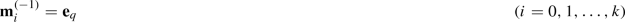(31a)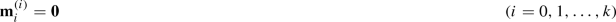(31b)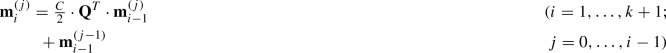(31c)

Then, $${\mathbf {m}}_{k+1}^{(0)},{\mathbf {m}}_{k+1}^{(1)},\ldots ,{\mathbf {m}}_{k+1}^{(k)}$$ satisfy the equations:

\begin{aligned} \left( j! \right) {\mathbf {m}}_{k+1}^{(j)}=\sum \limits _{i=0}^{k-j}{\tfrac{\left( i+j \right) !}{i!}{{(\tfrac{C}{2} {{{\mathbf {Q}}}^{T}})}^{i}}}{{{\mathbf {e}}}_{q}} \ \ (j=0,\ldots ,k) \end{aligned}
(32)

where x! denotes the factorial of x.

### Proof

When $$k=0$$, it follows from Eq. (31c) that

\begin{aligned} {\mathbf {m}}_{1}^{(0)}=\tfrac{C}{2}\cdot {{{\mathbf {Q}}}^{T}}\cdot \underbrace{{\mathbf {m}}_{0}^{(0)}}_{={\mathbf {0}}}+\underbrace{{\mathbf {m}}_{0}^{(-1)}}_{={{{\mathbf {e}}}_{q}}}={{{\mathbf {e}}}_{q}}. \end{aligned}

Thus, the following equation holds:

\begin{aligned} \left( 0! \right) \cdot {\mathbf {m}}_{1}^{(0)}={{{\mathbf {e}}}_{q}}=\sum \limits _{i=0}^{0}{\frac{(i+0)!}{i!}{{(\tfrac{C}{2}\cdot {{{\mathbf {Q}}}^{T}})}^{i}}}{{{\mathbf {e}}}_{q}} \ \ \text {(induction basis)} \end{aligned}

which implies that Eq. (32) holds for $$k=0$$. Assume that, for $$k=N$$, Eq. (32) holds, i.e.,

\begin{aligned} {\mathbf {m}}_{N+1}^{(j)}&=\frac{1}{j!}\sum \limits _{i=0}^{N-j}{\tfrac{\left( i+j \right) !}{i!}{{(\tfrac{C}{2}\cdot {{{\mathbf {Q}}}^{T}})}^{i}}}{{{\mathbf {e}}}_{q}} \quad \text {(hypothesis)} \nonumber \\&=\sum \limits _{i=0}^{N-j}{{i+j \atopwithdelims ()i}{{(\tfrac{C}{2} {{{\mathbf {Q}}}^{T}})}^{i}}}{{{\mathbf {e}}}_{q}} \ \ (j=0,\ldots ,N) \end{aligned}
(33)

We next show that, for $$k=N+1$$, Eq. (32) holds. Specifically, setting $$i=k \ (=N+1)$$ in Eq. (31c) produces

\begin{aligned} {\mathbf {m}}_{N+2}^{(j)}=\tfrac{C}{2}\cdot {{{\mathbf {Q}}}^{T}}\cdot {\mathbf {m}}_{N+1}^{(j)}+{\mathbf {m}}_{N+1}^{(j-1)}\quad (j=0,1,\ldots ,N+1) \end{aligned}

Plugging $${\mathbf {m}}_{N+1}^{(j)}$$ of Eq. (33) to the above equation yields

\begin{aligned}&{\mathbf {m}}_{N+2}^{(j)}=\tfrac{C}{2}\cdot {{{\mathbf {Q}}}^{T}}\cdot {\mathbf {m}}_{N+1}^{(j)}+{\mathbf {m}}_{N+1}^{(j-1)} \\&\quad =\sum \limits _{i=0}^{N-j}{ {i+j \atopwithdelims ()i} {{(\tfrac{C}{2}\cdot {{{\mathbf {Q}}}^{T}})}^{i+1}}}{{{\mathbf {e}}}_{q}} \\&\qquad +\sum \limits _{i=0}^{N-j+1}{ {i+j-1 \atopwithdelims ()i} {{(\tfrac{C}{2}\cdot {{{\mathbf {Q}}}^{T}})}^{i}}}{{{\mathbf {e}}}_{q}} \\&\quad =\sum \limits _{i=1}^{N-j+1}{{i+j-1 \atopwithdelims ()i-1} {{(\tfrac{C}{2}\cdot {{{\mathbf {Q}}}^{T}})}^{i}}}{{{\mathbf {e}}}_{q}} \\&\qquad +\left( {{{\mathbf {I}}}_{n}}+\sum \limits _{i=1}^{N-j+1}{ {i+j-1 \atopwithdelims ()i} {{(\tfrac{C}{2}\cdot {{{\mathbf {Q}}}^{T}})}^{i}}} \right) {{{\mathbf {e}}}_{q}} \\&\quad =\sum \limits _{i=1}^{N-j+1}{\underbrace{\left( {i+j-1 \atopwithdelims ()i-1} {+} {i+j-1 \atopwithdelims ()i} \right) }_{= {i+j \atopwithdelims ()i} } {{(\tfrac{C}{2} {{{\mathbf {Q}}}^{T}})}^{i}}}{{{\mathbf {e}}}_{q}}+{{{\mathbf {e}}}_{q}} \\&\quad =\sum \limits _{i=0}^{N+1-j}{ {i+j \atopwithdelims ()i} \cdot {{(\tfrac{C}{2}\cdot {{{\mathbf {Q}}}^{T}})}^{i}}}{{{\mathbf {e}}}_{q}} \end{aligned}

which completes the inductive step. $$\square$$

Leveraging Lemmas 2 and 3, we will complete the proof of Theorem 8.

### Proof of Theorem 8

Based on Lemma 2, setting $$i=k$$ in Eq. (30) produces

\begin{aligned} {{{\mathbf {u}}}_{k}}=\sum \limits _{j=0}^{k}{{{\left( \tfrac{C}{2}\cdot {\mathbf {Q}} \right) }^{j}}\cdot {\mathbf {m}}_{k+1}^{(j)}}. \end{aligned}
(34)

According to Lemma 3, $${\mathbf {m}}_{k+1}^{(0)},{\mathbf {m}}_{k+1}^{(1)},\ldots ,{\mathbf {m}}_{k+1}^{(k)}$$ defined by Eq. (28) satisfies

\begin{aligned} {\mathbf {m}}_{k+1}^{(j)}&=\sum \limits _{i=0}^{k-j}{\frac{\left( i+j \right) !}{i!j!}{{(\tfrac{C}{2}\cdot {{{\mathbf {Q}}}^{T}})}^{i}}}{{{\mathbf {e}}}_{q}} \nonumber \\&=\sum \limits _{i=0}^{k-j}{{i+j \atopwithdelims ()i}{{(\tfrac{C}{2}\cdot {{{\mathbf {Q}}}^{T}})}^{i}}}{{{\mathbf {e}}}_{q}}\quad (j=0,\ldots ,k) \end{aligned}
(35)

Substituting Eq. (35) into (34) produces

\begin{aligned} {{{\mathbf {u}}}_{k}}&=\sum \limits _{j=0}^{k}{{{\left( \tfrac{C}{2}\cdot {\mathbf {Q}} \right) }^{j}}\cdot \sum \limits _{i=0}^{k-j}{ {i+j \atopwithdelims ()i} {{(\tfrac{C}{2}\cdot {{{\mathbf {Q}}}^{T}})}^{i}}}{{{\mathbf {e}}}_{q}}} \nonumber \\&=\sum \limits _{j=0}^{k}{\sum \limits _{i=0}^{k-j}{{i+j \atopwithdelims ()i} \cdot {{\left( \tfrac{C}{2}\cdot {\mathbf {Q}} \right) }^{j}}{{(\tfrac{C}{2}\cdot {{{\mathbf {Q}}}^{T}})}^{i}}}}{{{\mathbf {e}}}_{q}} \nonumber \\&=\sum \limits _{l=0}^{k}{\sum \limits _{\alpha =0}^{l}{ {l \atopwithdelims ()\alpha } \cdot {{\left( \tfrac{C}{2}\cdot {\mathbf {Q}} \right) }^{\alpha }}{{(\tfrac{C}{2}\cdot {{{\mathbf {Q}}}^{T}})}^{l-\alpha }}}}{{{\mathbf {e}}}_{q}} \end{aligned}
(36)

The last equality holds since switching the order of the sum is equivalent (as pictorially depicted below):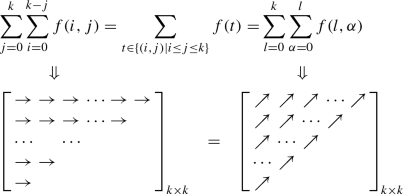Thus, plugging Eq. (36) into (26) produces

\begin{aligned} {{[{{\hat{\mathbf {S}}}_{k}}]}_{*,q}}&=(1-C)\cdot \sum \limits _{l=0}^{k}{ \frac{C^l}{2^l}\sum \limits _{\alpha =0}^{l}{{l \atopwithdelims ()\alpha } \cdot {{{\mathbf {Q}} }^{\alpha }} \cdot {{( {{{\mathbf {Q}}}^{T}})}^{l-\alpha }}}}{{{\mathbf {e}}}_{q}} \end{aligned}

Comparing this with the k-th partial sum of SimRank* in Eq. (14), we can see that our new iteration model in Eqs.(26)–(28) produces correct SimRank* results. $$\square$$

### 7.2 Single-source exponential SimRank*

Having derived the single-source geometric SimRank* model in Sect. 7.1, we next focus on the single-source exponential SimRank* assessment. To efficiently evaluate a single column of the exponential SimRank* matrix $$\hat{{\mathbf {S}}}_k'$$ in Eq. (16), we propose the following iterative model, whose CPU time and memory are not only linear w.r.t. the number of edges in the graph, but also less than those of the single-source geometric SimRank*.

### Theorem 10

(Single-Source Exponential SimRank*) Given query node q, the single-source exponential SimRank* between all nodes and q at the k-th iteration of Eq. (23), denoted as $${{[{{\hat{\mathbf {S}}}_{k}}']}_{*,q}}$$, can be iteratively derived as

\begin{aligned} {{[{{\hat{\mathbf {S}}'}_{k}}]}_{*,q}} = e^{-C} \cdot {{{\mathbf {v}}}_{k}} \end{aligned}
(37)

where the vector $${{{\mathbf {v}}}_{k}}$$ is iteratively derived by

\begin{aligned} \left\{ \begin{array}{l} {{{\mathbf {v}}}_{0}}={{{\mathbf {u}}}_{k}} \\ {{{\mathbf {v}}}_{i}}=\tfrac{C}{2(k-i+1)} {\mathbf {Q}} {{{\mathbf {v}}}_{i-1}} + {{{\mathbf {u}}}_{k}} \quad (i=1,2,\ldots ,k) \\ \end{array} \right. \end{aligned}
(38)

and the vector $${{{\mathbf {u}}}_{k}}$$ is iteratively obtained by

\begin{aligned} \left\{ \begin{array}{l} {{{\mathbf {u}}}_{0}}={\mathbf {e}}_{q} \\ {{{\mathbf {u}}}_{i}}=\tfrac{C}{2(k-i+1)} {\mathbf {Q}}^\mathrm{T} {{{\mathbf {u}}}_{i-1}} + {\mathbf {e}}_{q} \quad (i=1,2,\ldots ,k) \\ \end{array} \right. \end{aligned}
(39)

### Proof

We first prove that $${{{\mathbf {u}}}_{k}}=\sum _{j=0}^{k}{\tfrac{{{C}^{j}}}{{{2}^{j}}\cdot j!}{{({{{\mathbf {Q}}}^{T}})}^{j}}{{{\mathbf {e}}}_{q}}}$$.

Based on Eq. (39), for all $$i=1,2,\ldots ,k$$

\begin{aligned} {{{\mathbf {u}}}_{i}}-\tfrac{C}{2(k-i+1)}{{{\mathbf {Q}}}^{T}}{{{\mathbf {u}}}_{i-1}}={{{\mathbf {e}}}_{q}} \end{aligned}

Multiply both sides of this equation by $$\tfrac{{{C}^{k-i}}}{{{2}^{k-i}}\cdot (k-i)!}{{({{{\mathbf {Q}}}^{T}})}^{k-i}}$$, and then sum both sides from $$i=1$$ to k, which yields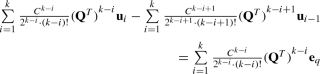(40)

Since

\begin{aligned} \text {LHS of (40)}&= \textstyle \sum \limits _{j=0}^{k-1}{\tfrac{{{C}^{j}}}{{{2}^{j}}\cdot j!}{{({{{\mathbf {Q}}}^{T}})}^{j}}{{{\mathbf {u}}}_{k-j}}}-\sum \limits _{l=1}^{k}{\tfrac{{{C}^{l}}}{{{2}^{l}}\cdot l!}{{({{{\mathbf {Q}}}^{T}})}^{l}}{{{\mathbf {u}}}_{k-l}}} \\&\textstyle ={{{\mathbf {u}}}_{k}}-\tfrac{{{C}^{k}}}{{{2}^{k}}\cdot k!}{{({{{\mathbf {Q}}}^{T}})}^{k}}{{{\mathbf {u}}}_{0}} \\ \text {RHS of (40)}&\textstyle = \sum \limits _{j=0}^{k-1}{\tfrac{{{C}^{j}}}{{{2}^{j}}\cdot j!}{{({{{\mathbf {Q}}}^{T}})}^{j}}{{{\mathbf {e}}}_{q}}} \end{aligned}

Thus,

\begin{aligned} {{{\mathbf {u}}}_{k}}&\textstyle =\tfrac{{{C}^{k}}}{{{2}^{k}}\cdot k!}{{({{{\mathbf {Q}}}^{T}})}^{k}}{{{\mathbf {u}}}_{0}}+\sum \limits _{j=0}^{k-1}{\tfrac{{{C}^{j}}}{{{2}^{j}}\cdot j!}{{({{{\mathbf {Q}}}^{T}})}^{j}}{{{\mathbf {e}}}_{q}}} \nonumber \\&\textstyle =\{\text {using } {{{\mathbf {u}}}_{0}}={{{\mathbf {e}}}_{q}} \} = \sum \limits _{j=0}^{k}{\tfrac{{{C}^{j}}}{{{2}^{j}}\cdot j!}{{({{{\mathbf {Q}}}^{T}})}^{j}}{{{\mathbf {e}}}_{q}}} \end{aligned}
(41)

Similarly, according to Eq. (38), we can prove that

\begin{aligned} \textstyle {{{\mathbf {v}}}_{k}}=\sum \nolimits _{l=0}^{k}{\tfrac{{{C}^{l}}}{{{2}^{l}}\cdot l!}{{{\mathbf {Q}}}^{l}}{{{\mathbf {u}}}_{k}}} \end{aligned}
(42)

Plugging Eqs.(41) and (41) into (37) produces

\begin{aligned} {{[{{\hat{\mathbf {S}}'}_{k}}]}_{*,q}}&\textstyle ={{e}^{-C}}\sum \limits _{l=0}^{k}{\tfrac{{{C}^{l}}}{{{2}^{l}}\cdot l!}{{{\mathbf {Q}}}^{l}} \bigg ( \sum \limits _{j=0}^{k}{\tfrac{{{C}^{j}}}{{{2}^{j}}\cdot j!}{{({{{\mathbf {Q}}}^{T}})}^{j}}{{{\mathbf {e}}}_{q}}} \bigg )} \\&={{e}^{-C}}{{e}^{\tfrac{C}{2}{\mathbf {Q}}}}{{e}^{\tfrac{C}{2}{{{\mathbf {Q}}}^{T}}}}{{{\mathbf {e}}}_{q}} \end{aligned}

$$\square$$

Theorem 10 implies an efficient algorithm, ss-eSR*, for single-source exponential SimRank* search. Its computational complexity is analyzed as follows:

### Theorem 11

(Complexity) Given a graph $${\mathcal {G}}$$, a query node q, and the total number of iterations K, ss-eSR* yields $$O(m+n)$$ memory and O(Km) time to iteratively compute single-source exponential SimRank* scores $${{[{{\hat{\mathbf {S}}'}_{K}}]}_{\star ,q}}$$.

### Proof

The memory of ss-eSR* is $$O(m+n)$$, which is dominated by (i) O(m) for storing sparse $${\mathbf {Q}}$$ (line 1), and (ii) O(n) for storing vectors $${\mathbf {u}}$$ (line 4) and $${\mathbf {v}}$$ (line 7).

The time complexity of ss-eSR* is O(Km), which is dominated by the matrix-vector multiplications $$({\mathbf {Q}}^\mathrm{T} \cdot {\mathbf {u}})$$ (line 4) and $$({\mathbf {Q}} \cdot {\mathbf {v}})$$ (line 7) for K iterations. $$\square$$

Compared with the $$O(K^2m)$$ time of the single-source geometric SimRank* algorithm ss-gSR*, the single-source exponential SimRank* reduces the time from $$O(K^2m)$$ to O(Km) further, linear with K. Moreover, the memory of ss-gSR* is improved from $$O(Kn+m)$$ to $$O(n+m)$$, independent of K. This is because, for the single-source exponential SimRank* computation, the iterative process in Eq. (38) relies only on the resulting $${\mathbf {u}}_K$$. Thus, there is no need of O(Kn) memory to store K vectors $$\{ {\mathbf {u}}_1, \ldots , {\mathbf {u}}_K \}$$ in Eq. (39).

### Example 4

Recall the graph in Fig. 1. Given query node b, the decay factor $$C=0.6$$, and the number of iterations $$k=3$$, the single-source exponential SimRank* $${{[{{\hat{\mathbf {S}}'}_{k}}]}_{*,b}}$$ can be computed via Theorem 10 as follows:

First, we iteratively obtain the auxiliary vector $${\mathbf {u}}_{3}$$ based on Eq. (39) as follows:

i

$${\mathbf {u}}_{i}$$

0

$${\mathbf {u}}_{0} = {\mathbf {e}}_{b}$$ $$={{[0, 1, 0, 0, 0, 0, 0, 0, 0, 0, 0]}^\mathrm{T}}$$

1

$${{{\mathbf {u}}}_{1}}=\tfrac{C}{2 \cdot 3} {\mathbf {Q}}^\mathrm{T} {{{\mathbf {u}}}_{0}} + {\mathbf {e}}_{b}$$ $$= [.1, 1, 0, 0, 0, 0, 0, 0, 0, 0, 0]^\mathrm{T}$$

2

$${{{\mathbf {u}}}_{2}}=\tfrac{C}{2 \cdot 2} {\mathbf {Q}}^\mathrm{T} {{{\mathbf {u}}}_{1}} + {\mathbf {e}}_{b}$$ $$= [.15,1, 0, 0, 0, 0, 0, 0, 0, 0, 0]^\mathrm{T}$$

3

$${{{\mathbf {u}}}_{3}}=\tfrac{C}{2 \cdot 1} {\mathbf {Q}}^\mathrm{T} {{{\mathbf {u}}}_{1}} + {\mathbf {e}}_{b}$$ $$= [.3, 1, 0, 0, 0, 0, 0, 0, 0, 0, 0]^\mathrm{T}$$

Next, we iteratively derive the vector $${\mathbf {v}}_3$$ from Eq. (38):

i

$${\mathbf {v}}_{i}$$

0

$${\mathbf {v}}_{0} = {{{\mathbf {v}}}_{3}}$$ $$=[.3, 1, 0, 0, 0, 0, 0, 0, 0, 0, 0]^\mathrm{T}$$

1

$${{{\mathbf {v}}}_{1}}=\tfrac{C}{2 \cdot 3} {\mathbf {Q}} {{{\mathbf {v}}}_{0}} + {{{\mathbf {u}}}_{3}}$$ $$=[.3, 1.03, .05, .015, .03, .1, .05, 0, .0333]^\mathrm{T}$$

2

$${{{\mathbf {v}}}_{2}}=\tfrac{C}{2 \cdot 3} {\mathbf {Q}} {{{\mathbf {v}}}_{1}} + {{{\mathbf {u}}}_{3}}$$ $$=[.3, 1.05, .078, .03, .045, .155, .078, .005, .054]^\mathrm{T}$$

3

$${{{\mathbf {v}}}_{3}}=\tfrac{C}{2 \cdot 3} {\mathbf {Q}} {{{\mathbf {v}}}_{2}} + {{{\mathbf {u}}}_{3}}$$ $$=[.3, 1.09, .161, .068, .09, .314, .161, .014, .112]^\mathrm{T}$$

Finally, $${{[{{\hat{\mathbf {S}}'}_{3}}]}_{*,b}}$$ can be obtained from $${{{\mathbf {u}}}_{3}}$$ via Eq. (37):

\begin{aligned}&{{[{{\hat{\mathbf {S}}'}_{3}}]}_{*,b}} = e^{-C} \cdot {{{\mathbf {u}}}_{3}} = e^{-0.6} \cdot {{{\mathbf {u}}}_{3}} \\&\quad = [.165, .598, .089, .037, .049, .172, .089, .007, .062]^\mathrm{T} \end{aligned}

$$\square$$

## 8 Comparison with “adding self-loops”

Apart from SimRank*, there is another simple method that adds a self-loop on each node of a graph to fix the “zero-similarity” issue of SimRank. In this section, we vindicate that SimRank* is more efficacious than the “adding self-loops” SimRank method in that there are many node-pairs over-counted in the similarity of the “adding self-loops” method.

To elaborate on this, we consider the first two consecutive steps of the two recursive models, respectively.

We first consider SimRank*. At the first step, $$\hat{s}(a,b)$$ is defined by the similarities between pairs of nodes:

\begin{aligned} \{(a',b)\}_{ a' \rightarrow a } \text { and } \{(a,b')\}_{b' \rightarrow b} \end{aligned}
(43)

Let us now unfold the SimRank* recursion one step further. We notice that (i) the similarity of $$(a',b)$$ is defined in terms of the similarity between pairs of nodes $$\{(a'', b') \}_{a'' \rightarrow a'}$$ and $$\{(a', b') \}_{a' \rightarrow a, b' \rightarrow b}$$; and (ii) the similarity of $$(a,b')$$ is defined in terms of the similarity between pairs of nodes $$\{(a', b')\}_{a' \rightarrow a, b' \rightarrow b}$$ and $$\{(a, b'')\}_{b'' \rightarrow b'}$$. Thus, at the second step, the SimRank* $$\hat{s}(a,b)$$ is defined in terms of the similarities between pairs of nodes:

\begin{aligned} \{(a'', b') \}_{a'' \rightarrow a'}, \{(a', b') \}_{a' \rightarrow a, b' \rightarrow b}, \{(a, b'')\}_{b'' \rightarrow b'} \end{aligned}
(44)

From (43) and (44), we see that there are no node-pairs repeatedly counted across the two consecutive steps of SimRank*.

In contrast, we next consider the “adding self-loops” method of SimRank. At the first step, after we add a self-loop on each node of the graph, SimRank defines the similarity between a pair of nodes (ab) in terms of the similarity between node-pairs:

\begin{aligned} \underline{\{(a', b') \}_{a' \rightarrow a, b' \rightarrow b}}, \{(a',b)\}_{ a' \rightarrow a }, \{(a,b')\}_{b' \rightarrow b} \end{aligned}
(45)

If we unfold the SimRank recursion one step further, we see that (i) the similarity of $$(a',b')$$ is defined in terms of the similarity between pairs of nodes $$\{(a'', b') \}_{a'' \rightarrow a'}$$, $$\{(a'', b'') \}_{a'' \rightarrow a', b'' \rightarrow b'}$$, and $$\{(a', b'') \}_{b'' \rightarrow b'}$$; (ii) the similarity of $$(a',b)$$ is defined in terms of the similarity between pairs of nodes $$\{(a'', b')\}_{a'' \rightarrow a', b' \rightarrow b}$$, $$\{(a'', b)\}_{a'' \rightarrow a'}$$ and $$\{(a', b')\}_{b' \rightarrow b}$$; and (iii) the similarity of $$(a,b')$$ is defined in terms of the similarity between pairs of nodes $$\{(a', b'')\}_{a' \rightarrow a, b'' \rightarrow b'}$$, $$\{(a', b')\}_{a' \rightarrow a}$$ and $$\{(a, b'')\}_{b'' \rightarrow b'}$$. Thus, at the second step, the similarity of the “adding self-loops” SimRank method is defined in terms of the similarities between pairs of nodes:

\begin{aligned}&\{(a'', b')\}_{a'' \rightarrow a', b' \rightarrow b}&\{(a'', b)\}_{a'' \rightarrow a'} \nonumber \\&\{(a', b'')\}_{a' \rightarrow a, b'' \rightarrow b'}&\{(a, b'')\}_{b'' \rightarrow b'} \nonumber \\&\underline{\{(a', b')\}_{a' \rightarrow a, b' \rightarrow b}} \end{aligned}
(46)

From (45) and (46), we notice that, for the “adding self-loops” method, the node-pairs $$\{(a', b')\}_{a' \rightarrow a, b' \rightarrow b}$$ (underlined parts) that have been counted in the first step are counted again in the next step. Over-counting the node-pairs $$\{(a', b')\}_{a' \rightarrow a, b' \rightarrow b}$$ will lead to excessive length weight coefficients assigned to the similarity contribution of the term $$\{(a', b')\}_{a' \rightarrow a, b' \rightarrow b}$$. In contrast, SimRank* has no over-counted node-pairs across two consecutive steps. Thus, the “adding self-loops” method of SimRank is less efficacious than SimRank*.

## 9 Experimental evaluation

### 9.1 Experimental settings

Datasets We adopt both real and synthetic datasets.

(1) Real datasets The size of each dataset is shown in Table 2. A detailed description is given in “Appendix E.1”.

(2) Synthetic datasets To produce synthetic networks, we use a graph generator GTgraphFootnote 3 that takes as input the number of nodes $$|{{\mathcal {V}}}|$$ and edges $$|{{\mathcal {E}}}|$$.

Compared algorithms We compare the following algorithms: (a) ss-gSR* and ss-eSR*, our single-source geometric and exponential SimRank* algorithms in Sect. 7; (b) SL-SR  and KM-SR , the state-of-the-art single-source SimRank algorithms based on indexing strategies and random sampling; (c) RWR , a fast random walk with restart algorithm measuring node proximities w.r.t. a given query; (d) memo-gSR* and memo-eSR*, the geometric and exponential SimRank* algorithms via partial sums memoization in Sect. 6; (e) psum-SR  and psum-PR , the SimRank and P-Rank algorithms via partial sums memoization; and (f) mtx-SR , a matrix-based method that computes Li et al. ’s SimRank using singular value decomposition.

Test queries For similarity ranking evaluation, we randomly select 500 query nodes from each dataset, based on the following: For each graph, we first sort all nodes in order of their importance (measured by PageRank) into 5 groups, and then randomly choose 100 nodes from each group, aiming to guarantee that the selected nodes can systematically cover a broad range of all possible queries.

Parameters We set the following default parameters: (a) $$C=0.6$$, the decay factor, as previously used in . (b) For all the iterative models, we set the number of iterations $$K=20$$ by default, to guarantee a high accuracy of $$C^K={0.6}^{21}\le 0.0000219$$. (c) For KM-SR, we follow the suggestion in , and set three parameters $$T=11$$, $$R=100$$, $$L=3$$, to ensure a worst-case error $$\epsilon =C^\mathrm{T}/(1-C)\approx 0.01$$. (d) For SL-SR, we follow Theorem 1 in , and set $$\epsilon _d = 0.003$$ and $$\theta = 0.0001$$, which guarantees its maximum error $$\epsilon < 0.01$$. We also set $$\delta _d = 1/n^2$$, which ensures that the preprocessing of SL-SR to achieve at least $$(1-1/n)$$ probability.

Effectiveness metrics To evaluate semantics and similarity ranking, we adopt the following three metrics: Kendall’s$$\tau$$, Spearman’s$$\rho$$, and Normalized Discounted Cumulative Gain (NDCG). Please refer to “Appendix E.2”.

Ground truth (a) To assess similar authors on DBLP, we invite 20 experts from database and data mining areas to verify the correctness of retrieved co-authorships. The experts have a strong research profile of international stature along with a sustained record of significant and world leading publications in databases/data mining areas, e.g., ACM TODS, VLDBJ, IEEE TKDE, ACM TKDD, SIGMOD, SIGKDD, PVLDB, ICDE. We selected the outstanding researchers with the combined expertise of data science from all over the world (e.g., USA, Europe, Australia, Asia) according to their Google Scholar profile with the minimum thresholds of $$\# \text {of citations}>1000$$ and $$\text {H-index}>20$$. Therefore, the selected scholars are familiar with their research domains, and can well evaluate relevant authors in data science through experience. They will also refer to “Co-Author Path” in Microsoft Academic SearchFootnote 4 to see “separations” between any two collaborators.

(b) To evaluate similar papers on CitH, we hire 15 researchers from the physical department to judge the “true” relevance of retrieved co-citations. The scholars have a proven track record of excellence in High Energy Physics research over the recent five years, with publications in e.g., Physical Review D, Nuclear Physics B, Journal of High Energy Physics, and Physics Letters B. We selected these scholars based on their productivity (number of high-quality publications) and research impact (number of citations) based on the Web of Science Core Collection (Thomson Reuters). These consistent publications in the high-impact journals indicate that the selected researchers have better knowledge in High Energy Physics research to well evaluate the similarities of papers in e-print arXiv. Their assessment may hinge on paper contents, H-index, and the number of citations in www.ScienceDirect.com. For all the ground truth, the results are rendered by a majority vote of feedbacks.

We use a computer powered by Intel Core i7-6700 3.40GHz CPU and 64GB RAM on Windows 8.

### 9.2 Experimental results

#### 9.2.1 Quantitative results on semantic effectiveness

We first run the algorithms on directed CitH and undirected DBLP. By randomly selecting 500 queries, we evaluate the average semantic accuracy for each algorithm via three metrics (Kendall, Spearman, NDCG). Figure 6a depicts the quantitative results. (1) On CitH, memo-gSR* and memo-eSR* have higher accuracy (e.g., Spearman’s $$\rho \approx 0.91$$) than psum-SR (0.29), RWR (0.12) and psum-PR (0.42) on average, i.e., the semantics of SimRank* is effective. This is because SimRank* considers all in-link paths for assessing similarity, whereas SimRank and RWR, respectively, counts only limited symmetric and unidirectional paths. (2) On DBLP, the accuracy of RWR is the same as memo-gSR* and memo-eSR*, due to the undirectedness of DBLP. This tells us that, regardless of edge directions, both SimRank* and RWR count the path of all lengths, as opposed to SimRank considering only the even-length paths. Likewise, psum-PR and psum-SR produce the same results on undirected DBLP. (3) On each dataset, memo-gSR* and memo-eSR* keep almost the same accuracy, implying that the relative order of the geometric SimRank* is well maintained by its exponential counterpart.

Figure 6b shows the “zero-similarity” issues commonly exist in real graphs for JSR, LSR, RWR, ASCOS. (1) On CitH, $$\sim \,$$97.9% node-pairs have “zero-SimRank” problems for both JSR and LSR, among which 19.2% pairs (resp. 78.7%) have “completely dissimilar” (resp. “partially missing”) issues whose similarities are 0s (resp. not 0s but neglect the contributions of asymmetric paths). Similarly, on CitH, $$\sim \,$$94.1% pairs have “zero-similarity” issues for both RWR and ASCOS, highlighting the seriousness of this problem. (2) On D09, almost 99.99% pairs have “partially missing zero-similarity” issues for each similarity measure despite very little “completely dissimilar” issues, due to the undirectness of DBLP. (3) The amount of “zero-similarity” pairs evaluated by JSR (resp. RWR) is the same as that by LSR (resp. ASCOS). This is consistent with our analysis in Corollary 2.

#### 9.2.2 Qualitative case studies on semantics

Figure 7 presents the case study of qualitative results for top-k similarity ranking w.r.t. queries Q1–Q4 on DBLPD09 (2009–2011). For example, Q1 finds the most similar co-authors of Prof. Jennifer Widom, by using different similarity measures, e.g., SimRank* (memo-gSR*, memo-eSR*), Random Walk with Restart (RWR), SimRank without adding self-loops (psum-SR), and SimRank by adding self-loops (self-loop). We observe that (1) RWR and memo-gSR* produce the same results on DBLP, which is due to the undirectedness of DBLP, as expected. (2) memo-gSR* and memo-eSR* also yield the same results for our top-k similarity search, showing the relative ranking preservation of memo-eSR* w.r.t. memo-gSR*. (3) Some close co-authors of Prof. Jennifer Widom that are ranked lower undesirably by psum-SR (as shown in the brackets of the gray cells) can be well identified by memo-gSR*, memo-eSR*, and RWR. For instance, “Anish Das Sarma”, who has many collaborative publications with Prof. Jennifer Widom during 2009–2011, is ranked among top 5 by memo-gSR* and memo-eSR*, but not top ranked by psum-SR and self-loop. This is because SimRank ignores the contributions of asymmetric in-link paths (i.e., the paths of odd lengths in undirected graphs), whereas SimRank* considers the contributions of all in-link paths. As a result, many close co-authors (with high degrees of one-edge connection) of Prof. Jennifer Widom (e.g., Dr. Anish Das Sarma) are missed by SimRank, but can be found effectively by SimRank*. The disparity of ranking in gray cells shows that memo-gSR*, memo-eSR*, RWR can perfectly resolve the “zero-similarity” issue of psum-SR on undirected graphs. (4) self-loop is more effective than SimRank, but sometimes less effective than SimRank*. For example in Q1, “Huacheng C. Ying” and “Qi Su” are identified by both SimRank* and self-loop, but they are ignored by SimRank. However, “Anish Das Sarma”, Prof. Jennifer Widom’s student, is not captured by SimRank or self-loop. “Beverly Yang” is ranked at $$6^{\text {th}}$$ by self-loop, but he has no collaborative publications with Prof. Jennifer Widom on DBLP (2009–2011). This is due to the over-counting problem of self-loop that will lead to excessive length weight coefficients counter-intuitively assigned to the pair (“Beverly Yang” and “Prof. Jennifer Widom”). In some cases, self-loop achieves the ranking results as good as SimRank*. For instance in Q4, the top-4 most similar author-pairs in D09 (2009–2011) by SimRank* and self-loop are the same, both of which are more reliable than SimRank as they do not have “zero-SimRank” issues.

We next provide some qualitative results on the directed graph CitH. The similarity ranking results w.r.t. three paper queries are shown in Fig. 8. It can be noted that (1) for directed CitH, RWR and memo-gSR* have substantial differences. For the first query Q1, the top-4 ranking results identified by RWR are not the most relevant articles w.r.t. the query article. This is because RWR considers only unidirectional paths between two nodes, thus limiting its utility for find sensible papers, whereas SimRank* considers all in-link paths. Other results on SimRank* and SimRank are analogous to those on DBLP. (2) The semantics of SimRank* is more effective than those of SimRank and self-loop. For example in Q1, consider the two most similar articles retrieved by SimRank* (i.e., “Probing Solitons in Brane Worlds” and “Localization of Bulk Form Fields on Dilatonic Domain Wall” highlighted in the light gray cells). SimRank captures only the first one, and self-loop only the second one, but they are unable to capture both. The reason is that SimRank will neglect the contributions of asymmetric in-link paths, whereas self-loop will overcount the contributions of symmetric in-link paths. Both of them will produce the biased similarity ranking results. In contrast, SimRank* retrieves the most appropriate articles by considering both symmetric and asymmetric in-link paths with reasonable weighted coefficients, whose results are better than SimRank and self-loop.

#### 9.2.3 Scalability of ss-eSR* and ss-gSR*

To evaluate the scalability of SimRank* on large graphs, we compare the computational time and memory space of ss-eSR* and ss-gSR* with other algorithms on various real datasets with m ranging from 17 K to 1.15 G. We randomly select 20 queries, Q, from each dataset, and retrieve all the similarities $$\{s(*,q)\}_{q \in Q}$$. Note that our query selection is based on its node PageRank value so that Q can cover a board range of queries. Figure 9 depicts the results for $$K=20$$.

We notice that (1) memo-gSR*, psum-SR, and mtx-SR only survive on small-scale datasets (e.g., DBLP and CitH). For large-scale datasets, ss-eSR*, ss-gSR*, KM-SR, RWR scale well. The in-memory version of KM-SR will explode on billion-scale UK05 and IT04, due to its huge space cost for indexing. (2) On each dataset, ss-eSR* and RWR are faster than the other algorithms as they only require linear time w.r.t. the number of edges and K. To attain the same accuracy, the query time of SL-SR and KM-SR is much faster than ss-eSR* (see Fig. 10), but the total time of SL-SR and KM-SR is 6–9$$\times$$ larger than that of ss-eSR* and ss-gSR*. This is because SL-SR and KM-SR spend a large amount of time building index for preprocessing (see Fig. 10), whereas ss-eSR* and ss-gSR* are non-indexing algorithms. Thus, when the number of queries is not large, ss-eSR* and ss-gSR* are more time-efficient. When the number of queries becomes large, e.g., $$|Q|=n$$, the total time of SL-SR and KM-SR can be faster than ss-eSR* and ss-gSR*, but are slower than memo-eSR* and memo-gSR* algorithms. (3) On small datasets (e.g., DBLP and CitH) when memo-gSR* and psum-SR do not fail, ss-eSR* and ss-gSR* are 2.5–3 orders of magnitude faster than memo-gSR* and psum-SR. The reason is that, given queries, ss-eSR* and ss-gSR* can compute similarities on an as-needed basis, as opposed to memo-gSR* and psum-SR that are query-independent and always output all-pairs similarities. (4) The memory space of ss-eSR* and ss-gSR* is 2–3 orders of magnitude less than that of memo-gSR* and psum-SR, highlighting its scalability on billion-scale graphs. (5) The memory of KM-SR and RWR is comparable to that of ss-eSR* and ss-gSR*, all of which have less space than SL-SR. This is consistent with our space complexity analysis. The extra memory of SL-SR is due to its storage for indexing structures.

#### 9.2.4 Varying |Q| for ss-gSR* and ss-eSR*

To evaluate the effect of query size |Q| on the computational efficiency of ss-eSR* and ss-gSR*, we fix $$K=20$$ and vary |Q| from 200 to 600 on D02 and CitH, and compare the computation time and memory space of ss-gSR* with memo-gSR*, and ss-eSR* with memo-eSR*. The results on D02 and CitH are shown in Figs. 11 and 12, respectively. Since memo-gSR* will fail on large datasets, we vary |Q| from 10 to 200 on WebB, WikT, SocL, and show the CPU time and memory of ss-gSR* and ss-eSR* in Figs. 13 and 14, respectively.

From the results, we notice that (1) when |Q| grows from 200 to 600, the time of ss-eSR* and ss-gSR* increase linearly on both D02 and CitH, whereas the time of memo-eSR* and memo-gSR* are insensitive to |Q|, remaining at constant time on D02 and CitH, respectively. This conforms to our expectation as ss-eSR* and ss-gSR* adopt novel iterative models that provide on-demand retrieval w.r.t. given queries. In contrast, memo-eSR* and memo-gSR* are query-independent algorithms which have to assess all-pairs similarities simultaneously even if one wishes only a fraction of pairs of similarities. (2) As |Q| increases on D02 and CitH, the memory of all the algorithms remains unaltered, insensitive to the query size. The reason is that, for each single-source query q, ss-gSR* will immediately release the auxiliary vector $${\mathbf {m}}_{i-1}^{(j-1)}$$ when it has been used twice for iteratively generating the Pascal’s triangle pattern; after each query q, ss-gSR* will also release the memory to start with a new retrieval w.r.t. another single-source query $$q'$$. For ss-eSR*, in each query q, only one auxiliary vector needs memoization after each iteration. The memory space of memo-eSR* and memo-gSR* is always dominated by $$O(n^2)$$ to store all-pairs similarities regardless of query size, and thereby remains constant as |Q| varies. (3) On large datasets (e.g., WebB, WikT, SocL) in Figs. 13 and 14, when |Q| varies from 10 to 200, the time and memory of ss-eSR* and ss-gSR* exhibit a similar tendency to those on small datasets (D02 and CitH), indicating that ss-eSR* and ss-gSR* scale well to both the graph size and the query size |Q|.

#### 9.2.5 Varying K for ss-gSR* and ss-eSR*

Finally, we evaluate the effect of the number of iterations, K, on the computational time and memory of ss-gSR* and ss-eSR*. Fixing the query size $$|Q|=100$$, we vary K from 10 to 40 on three large datasets (WebB, WikT, SocL), respectively. The results are shown in Figs. 15 and 16. It can be discerned that (1) given $$|Q|=100$$, when K grows, the computational time of both ss-gSR* and ss-eSR* increases on every dataset. ss-gSR* increases dramatically, whereas ss-eSR* grows mildly. This is in accord with our time complexity bound analysis in Sect. 7, in which the time of ss-gSR* is quadratic w.r.t. K, whereas the time of ss-eSR* is linear w.r.t. K. (2) For any fixed |Q|, the memory of ss-gSR* increases mildly as K grows, but the memory of ss-eSR* remains unchanged as K increases. This is because ss-gSR* requires O(Kn) memory for storing $$(K+1)$$ auxiliary vectors $$\{{\mathbf {m}}_{K+1}^{(0)}, \ldots , {\mathbf {m}}_{K+1}^{(K)}\}$$ to iteratively retrieve SimRank*, whereas ss-eSR* needs O(n) memory to store one auxiliary vector from the previous iteration, which is independent of K. This agrees well with our space complexity analysis of ss-gSR* in Theorem 9, and ss-eSR* in Theorem 11.

## 10 Related work

One of the most attractive link-based similarity measures is SimRank, proposed by Jeh and Widom . The recursive nature of SimRank allows two nodes to be similar even without common in-neighbors sharing, which resembles PageRank  that recursively assigns a score for node ranking. However, SimRank implies some unsatisfactory traits. One limitation is that “the similarity of two nodes will decrease as the number of their common in-neighbors increases”. To address this problem, many excellent methods have been proposed, leading to several SimRank variant models. For example, Fogaras and Rácz  introduced P-SimRank. They (1) incorporated Jaccard coefficients, and (2) interpreted s(ab) as the probability that two random surfers, starting from a and b, will meet at a node. Antonellis et al.  proposed SimRank++, by adding an evidence weight to compensate for the cardinality of in-neighbor matching. Lin et al.  presented MatchSim, which refines SimRank with maximum neighborhood matching. Jin et al.  proposed RoleSim that generalizes Jaccard coefficients to ensure automorphic equivalence for SimRank. Yu and McCann et al.  introduce SimRank#, a high-quality SimRank-based model that extends cosine similarity measure to aggregate pairs of multi-hop paths.

Another limitation of SimRank is the “zero-similarity” problem that “$$s(a,b)=0$$ if there are no nodes having equal distance to both a and b”. A special case of this problem was observed by Zhao et al. [36, Example 1.2]. They proposed P-Rank by taking both in- and out-links into account. P-Rank indeed can reduce the number of pairs of nodes with counter-intuitive zero similarities. However, if there are neither equidistant in-link paths nor equidistant out-link paths from two nodes a and b, the similarity of (ab) is still zero. Our work is different from  in that (1) we show that the “zero-SimRank” problem is not caused by the ignorance of out-links in SimRank, and (2) we circumvent the “zero-similarity” issue by traversing more incoming paths of node-pairs that are neglected by the original SimRank. Recently, Chen and Giles  also proposed a similarity model, ASCOS++, to address the SimRank issue that “if the length of a path between two nodes is an odd number, this path makes no contribution to the SimRank score”. The issue is a special case of our “zero-similarity” issue. It differs from our work in that  provided a sufficient condition for $$s(a,b)=0$$, whereas we give a sufficient and necessary condition for $$s(a,b)=0$$. That is, “the odd-length path between two nodes a and b” given by  is not the only condition that will lead to $$s(a,b)=0$$. Another condition that “the even-length in-linked paths between nodes a and b whose ‘source’ node is not in the center of the path” also leads to $$s(a,b)=0$$. Therefore, ASCOS++ only partially resolved our “zero-similarity” issue of SimRank, as we discussed in Sect. 3.5.

There has also been research on link-based similarity (e.g., [4, 18, 28,29,30]). LinkClus  adopted a hierarchical structure, called SimTree, for clustering multi-type objects. Blondel et al.  proposed an appealing measure to quantify graph-to-graph similarity. SimFusion  exploited a reinforcement assumption to assess similarities of multi-type objects in a heterogenous domain, as opposed to SimRank focusing solely on intra-type objects in a homogenous domain. Tong et al.  suggested Random Walk with Restart (RWR) for assessing node proximities, which is an excellent extension of Personalized PageRank (PPR). Leicht et al.  extend RWR by incorporating independent and sensible coefficients. However, RWR and its variants (PPR and ) also imply SimRank-like “zero-similarity” issues, as discussed in Sect. 3.4. The recent work of [16, 34] has showed that Jeh and Widom’s SimRank model  and Li et al. ’s SimRank model  are different. In the previous conference version , we only proved the existence of “zero-similarity” issues in Li et al. ’s SimRank model . In this work, we show further that “zero-similarity” issues also exist in Jeh and Widom’s SimRank model . Moreover, we prove in Sect. 3.3 that the affected pairs of nodes in these two SimRank models are exactly the same.

### 10.2 Optimization methods for computing similarities

The computational overheads of SimRank-based similarity arise from its recursive nature. To reduce its computational complexity, a number of efficient techniques have been proposed to optimize SimRank computation, including all-pairs search, single-source search, single-pair search, and partial-pairs search.

For all-pairs search, Lizorkin et al.  focused on SimRank iterative computation and proposed three excellent optimization approaches (i.e., essential node-pair selection, partial sums memoization, and threshold-sieved similarities). These substantially speed up SimRank computation from $$O(Kd^2n^2)$$ to O(Knm) time. Later, Yu et al.  used a mini spanning tree to find the topological sort for fine-grained partial sums sharing, which improved all-pairs SimRank search further to $$O(Kd'n^2)$$ time (with $$d' \le d$$). However, both methods require $$O(n^2)$$ memory to output all-pairs results at each iteration, which are impractical to large-scale graphs. Li et al.  developed a SVD-based SimRank matrix computing model to approximate SimRank results, yielding $$O(r^4 n^2)$$ time, where $$r \ (\le n)$$ is the targeted rank of SVD. However, it does not always speed up the computation when r is large for achieving a high accuracy. In contrast, our SimRank* model is fast and memory-efficient. It scales well on billion-edge graphs while tallying even more paths than SimRank to enrich semantics.

For single-source search, Lee et al.  first proposed a pioneering model, TopSim, that used a Monte Carlo method to retrieve top-k SimRank pairs in $$O(d^k)$$ time. To trade accuracy for speed, they also presented two approximate techniques based on truncated random walk and prioritizing propagation, respectively. Later, Fujiwara et al.  presented SimMat, which (1) retrieves the top-k similar nodes based on a Sylvester equation, and (2) prunes unnecessary search based on the Cauchy-Schwarz inequality. Kusumoto et al.  introduced a “linear” recursive formula for SimRank, based on which they establish a novel random-walk-based method for scalable top-k single-source similarity search. Tian and Xiao  designed an efficient index structure, SLING, for SimRank search that guarantees the worst-case error in each SimRank score returned. Recently, Shao et al.  and Jiang et al.  devised TSF and READS indexing schemes, respectively, to efficiently handle top-k SimRank search over dynamic graphs. Liu et al.  presented ProbeSim, an index-free solution for dynamic single-source and top-k SimRank queries with provable accuracy guarantees.

There has also been other work on SimRank search. Fogaras and Rácz  proposed P-SimRank for a single-pair SimRank retrieval. Li et al.  developed CloudWalker, a parallel algorithm for large-scale SimRank search on Spark with ten machines. Tao et al.  proposed an excellent two-stage way for the top-k SimRank-based similarity join. Zhang et al.  conducted comprehensive experiments and compare many existing SimRank algorithms in a unified environment. Their empirical study showed that, despite recent research efforts, the computational time and precision of known algorithms have still much space for improvement.

## 11 Conclusions

In this article, we have proposed SimRank*, an effective and scalable similarity model, for effectively assessing link-based similarities. In contrast to SimRank that considers only the contributions of symmetric in-link paths, SimRank* tallies the contributions of all in-link paths between two nodes, thus resolving the “zero-SimRank” issue for semantic richness. We have also converted the series form of SimRank* into two elegant forms: the geometric SimRank* and its exponential variant, both of which look even simpler than SimRank, yet without suffering from increased computational cost. To speedup all-pairs SimRank* search, we have devised a fine-grained memoization strategy via edge concentration, with an efficient algorithm speeding up SimRank* computation from O(Knm) to $$O(K n{\tilde{m}})$$ time, where $${\tilde{m}}$$ is generally much smaller than m. However, the memory of this algorithm is still $$O(n^2)$$, which is not applicable to sizable graphs. To scale SimRank* on billion-edge graphs, we propose two memory-efficient single-source algorithms, ss-gSR* for geometric SimRank* search, and ss-eSR* for exponential SimRank* search without any loss of accuracy. ss-gSR* utilizes a Pascal’s triangle pattern that requires $$O(K^2 {\tilde{m}})$$ time and $$O(Kn + {\tilde{m}})$$ memory to iteratively retrieve SimRank* similarities between all n nodes and a given query on an as-needed basis, whereas ss-eSR* employs a novel iterative model that entails only $$O(K {\tilde{m}})$$ time and $$O(n + {\tilde{m}})$$ memory, where $${\tilde{m}} \ll n^2$$. We also compare SimRank* with another alternative remedy for SimRank that adds self-loops on each node, and vindicate that SimRank* is more efficacious. Our experimental results on real and synthetic data demonstrate the richer semantics, higher computational efficiency, and scalability of SimRank* on billion-scale graphs.